# ICSE Selina Solution for Class 9 Chemistry Chapter 7

ICSE Selina Solution for Class 9 Chemistry Chapter 7 given in this document serves as the best study material for ICSE class 9 Chemistry exam preparation. This page has answers to all the exercise questions provided in the Selina publishers class 9 Chemistry textbook. Answers provided here are written by highly experienced teachers at BYJU’S.

ICSE Selina solutions for class 9 will help you lay a strong foundation of the concepts involved in class 9 syllabus. Detailed answers provided here will help to clear all the concepts involved in the syllabus. Exercise questions have MCQ’S, Short and long answer questions, numerical problems and questions based on formula derivations which are very important from the point of examinations.

Chapter 7 Study of Gas Laws is an important chapter of ICSE class 9 Chemistry syllabus, as it carries a good weightage of marks in the examination. This chapter also serves as the basic chapter required to study Physical Chemistry topics in future. This document has a variety of questions like MCQ, filling the blanks, questions based on derivations and numerical problems.

ICSE Selina solutions for class 9 chemistry provided here explains the behaviour of gases under different temperatures and pressure. This solution page has questions regarding Charles law, Boyle’s law, mathematical calculation involved in Charle’s and Boyle’s law, the relationship between Kelvin and Celsius scale of temperature and their conversions.

### Download PDF of ICSE Selina Solution for Class 9 Chemistry Chapter 7 Study of Gas Laws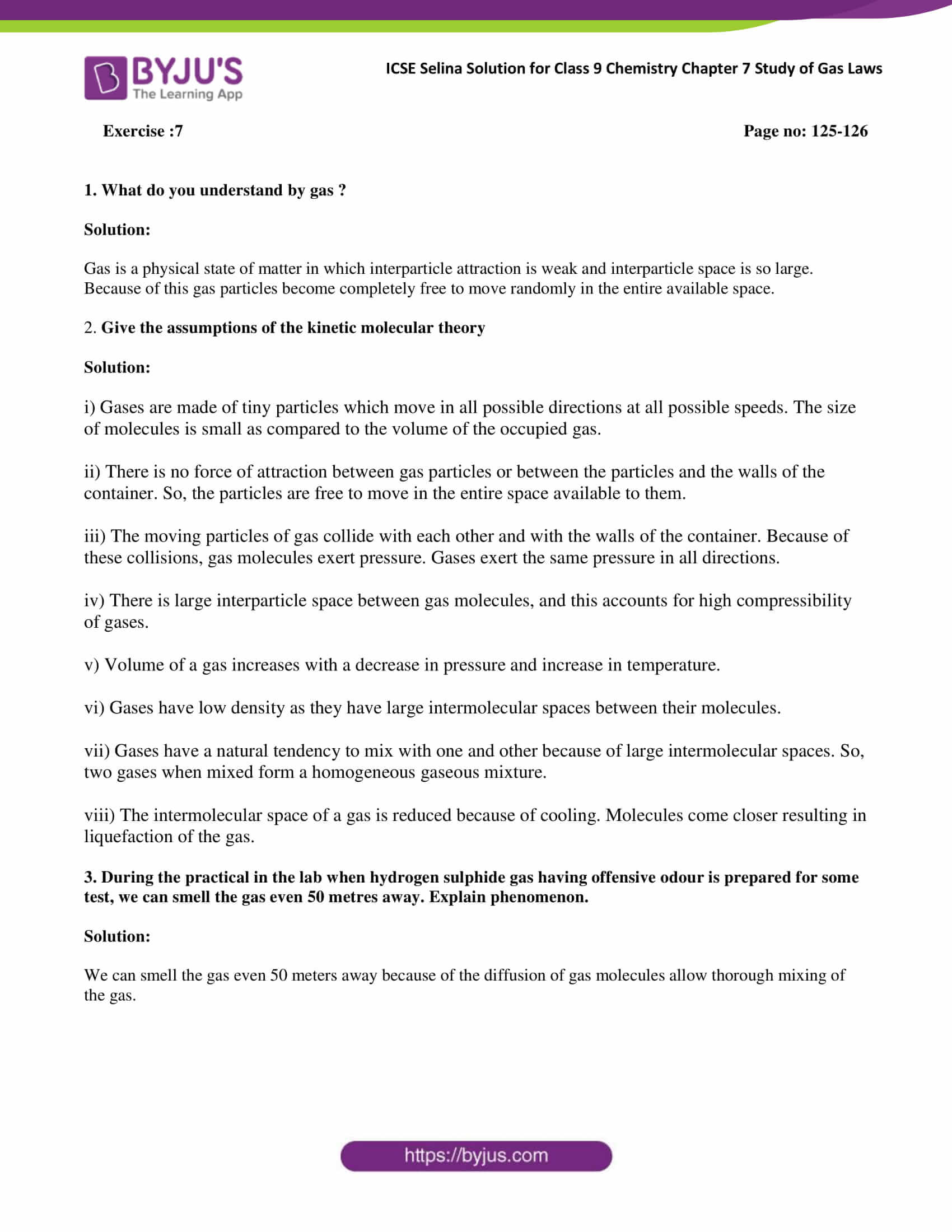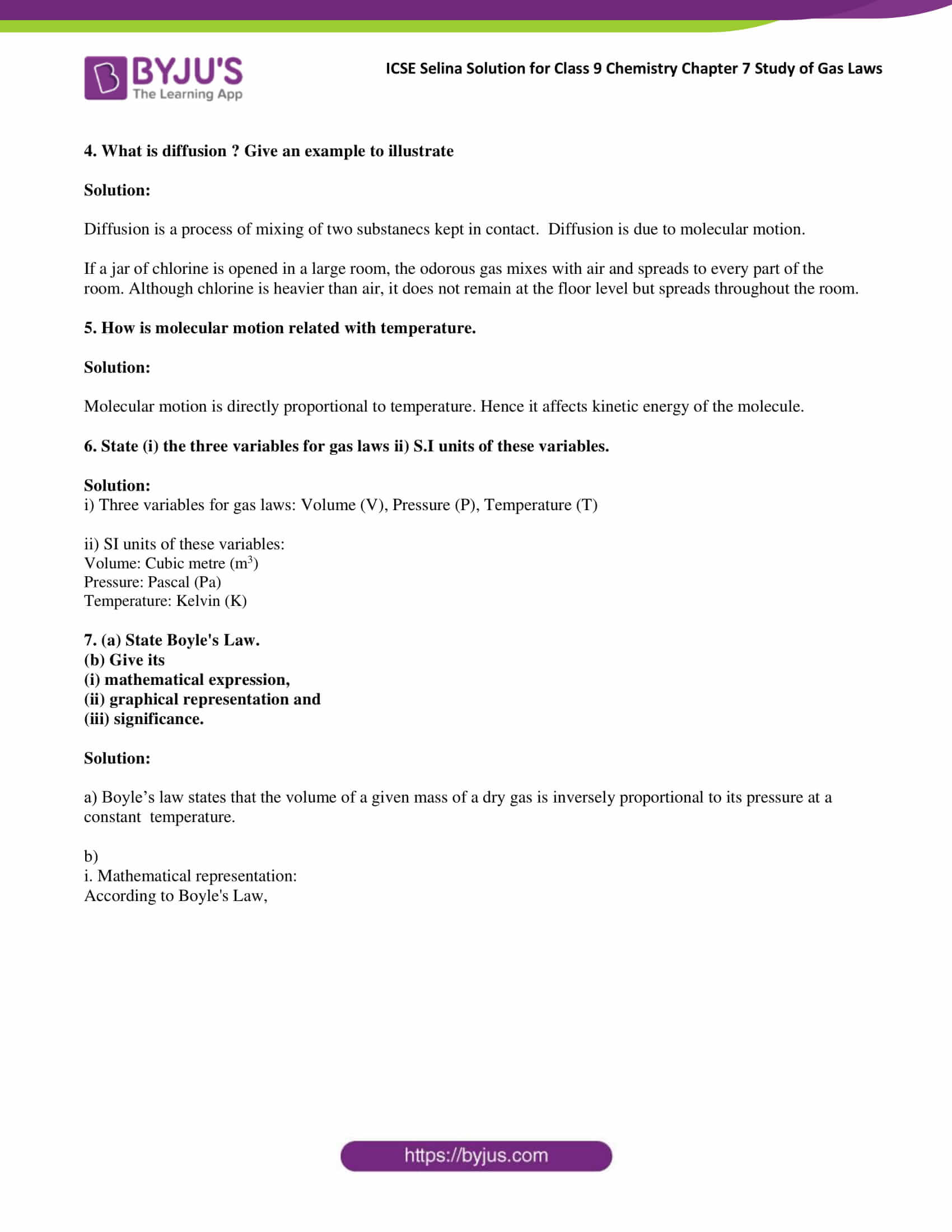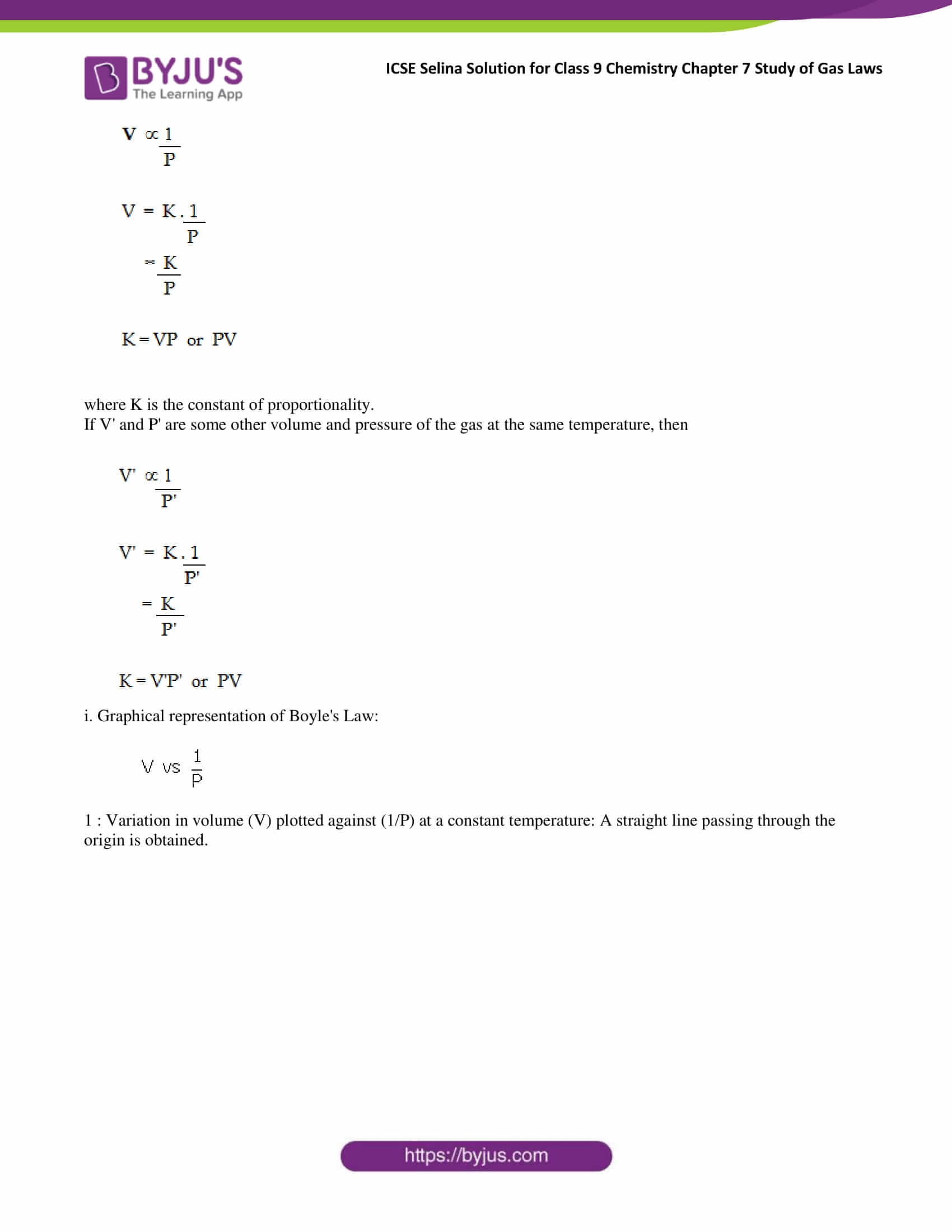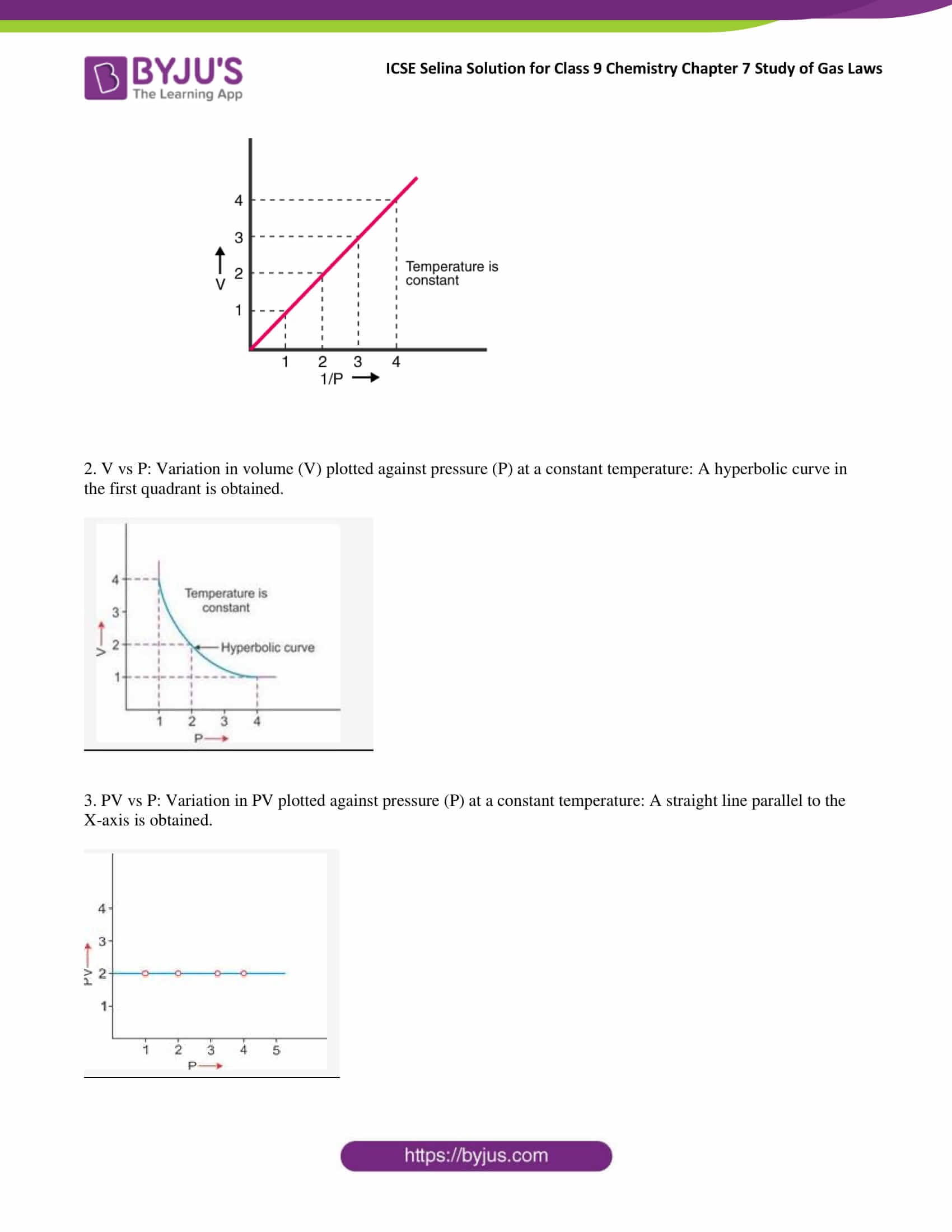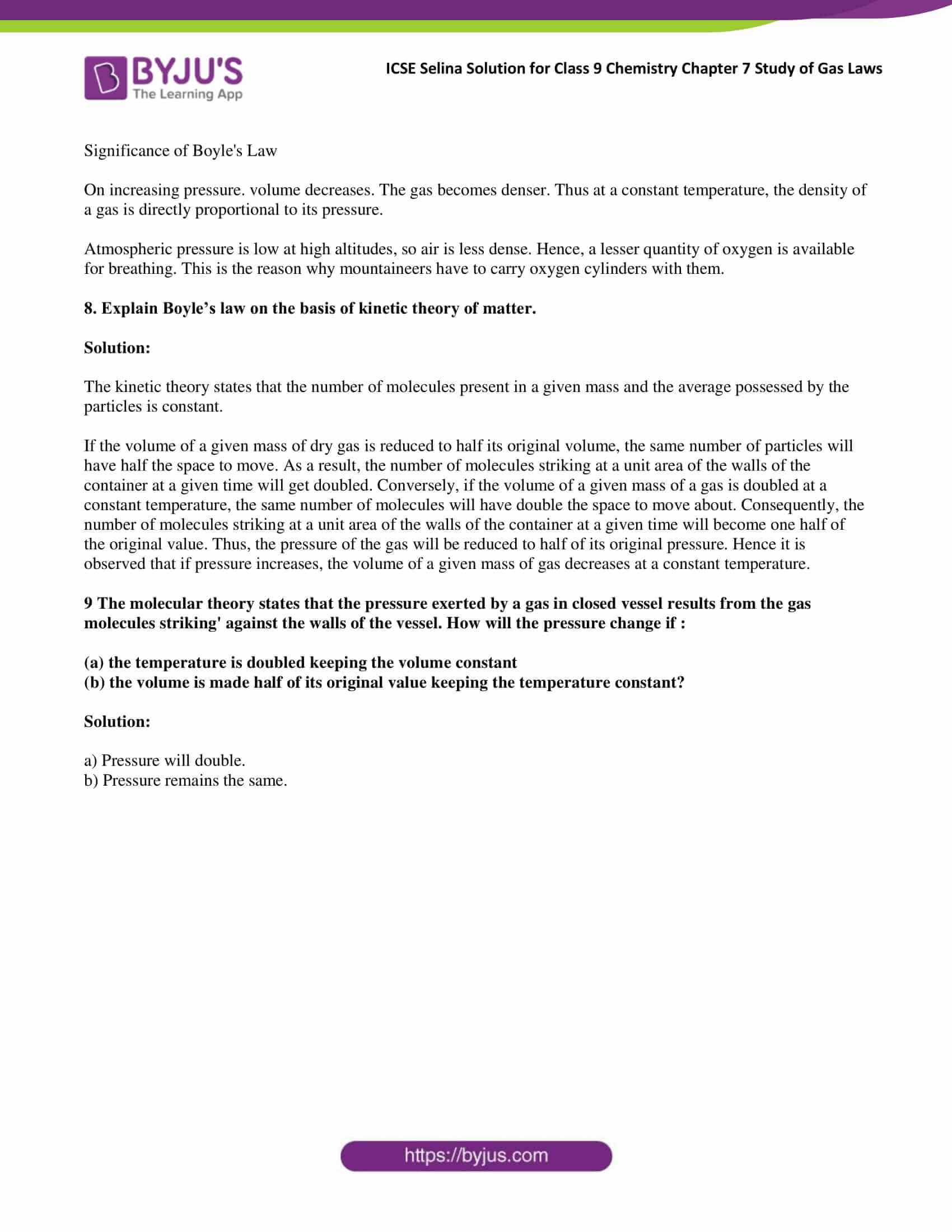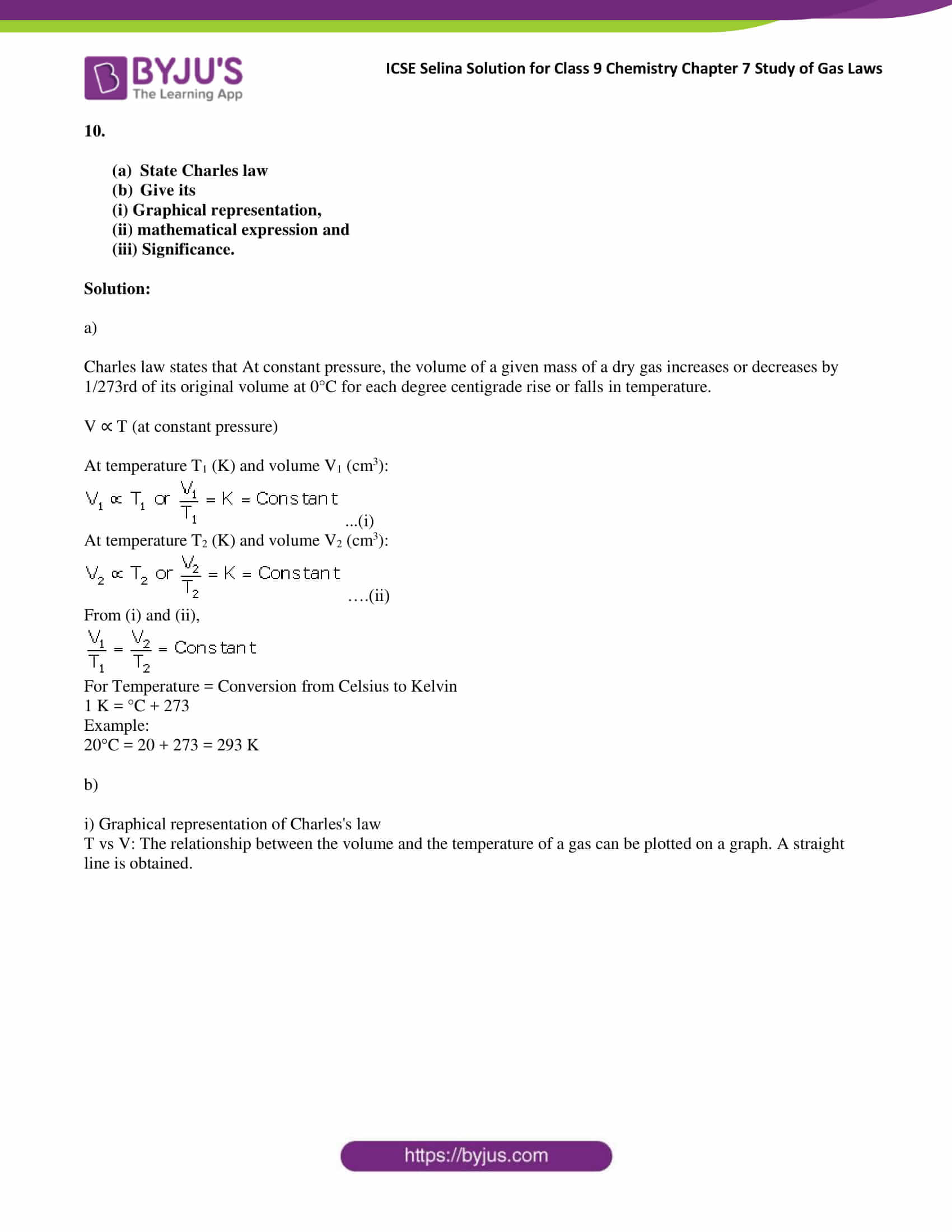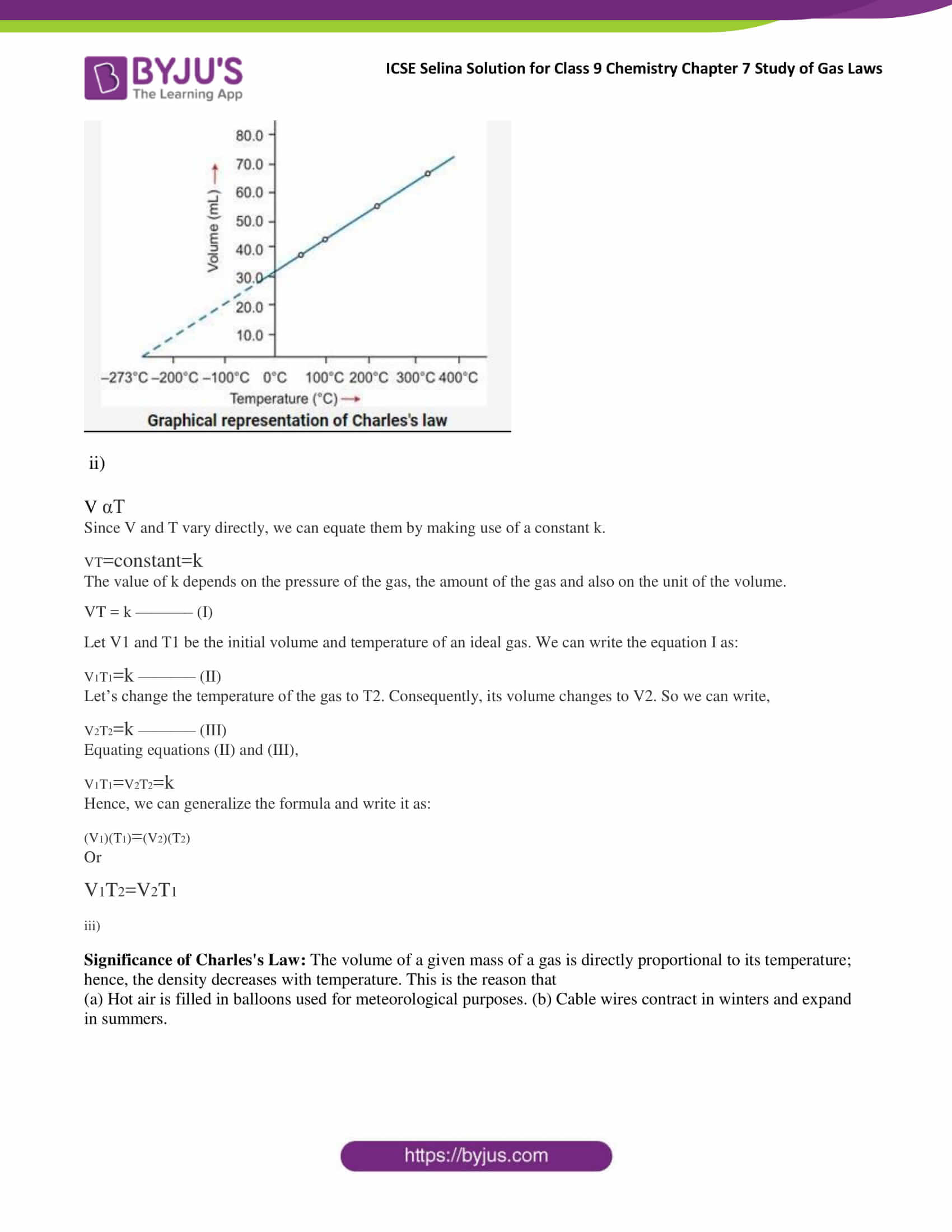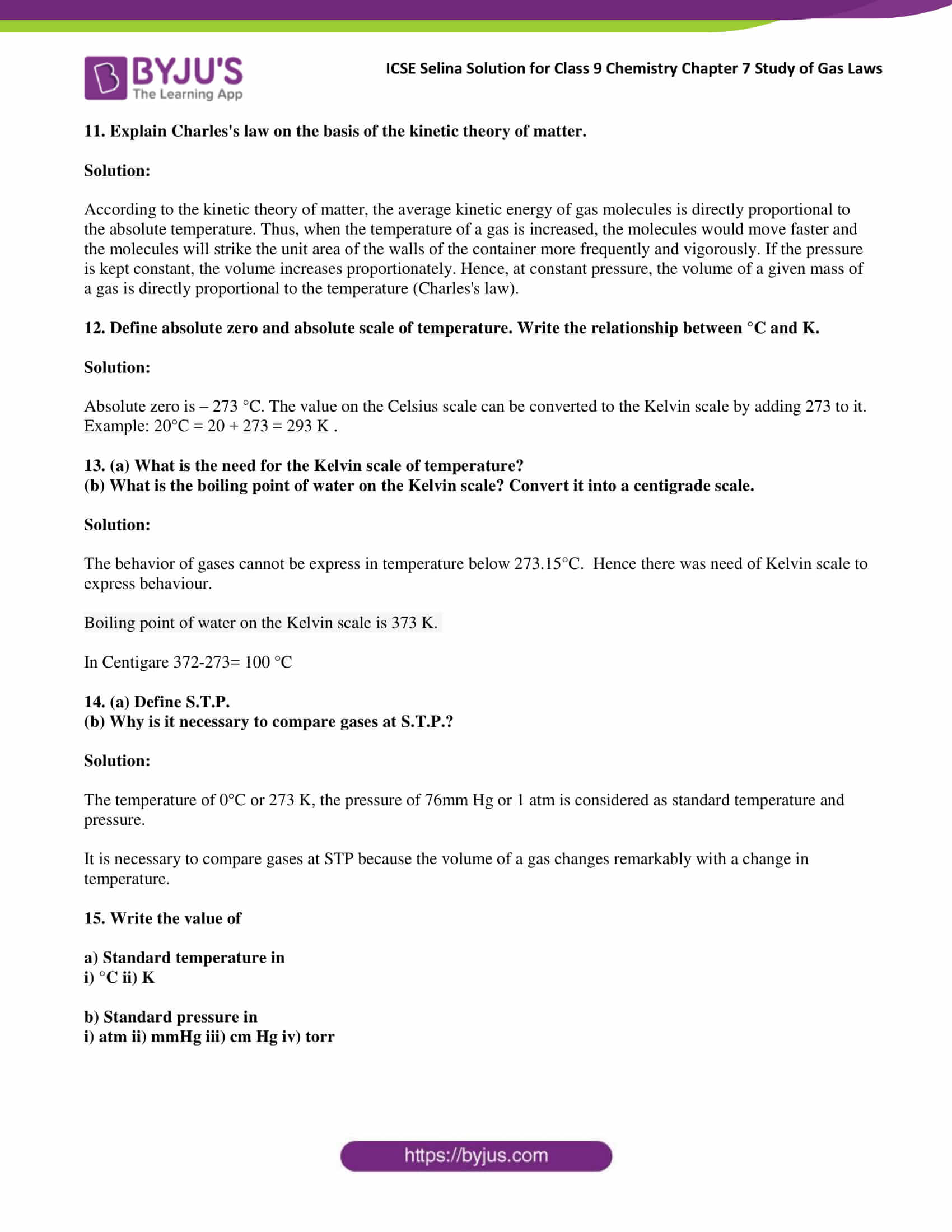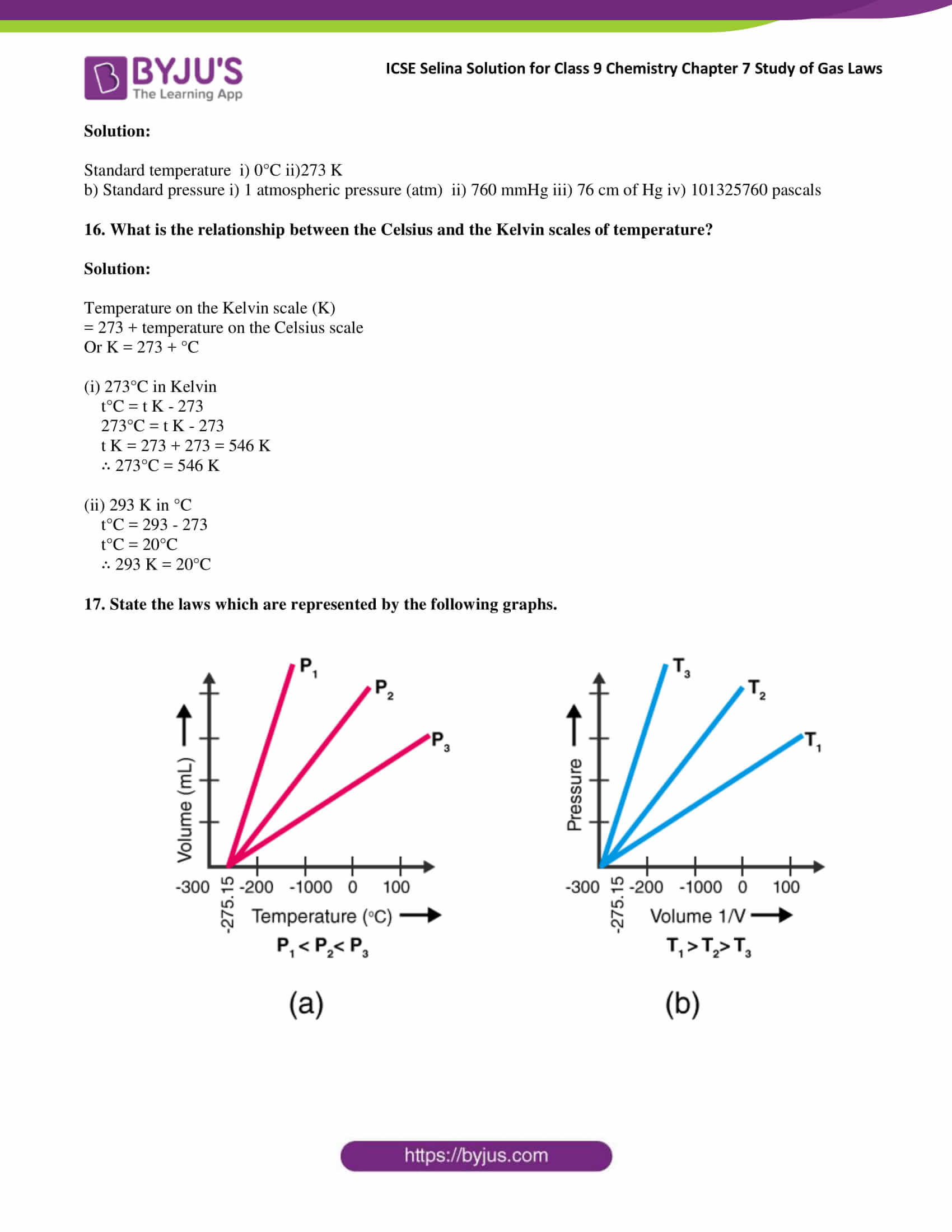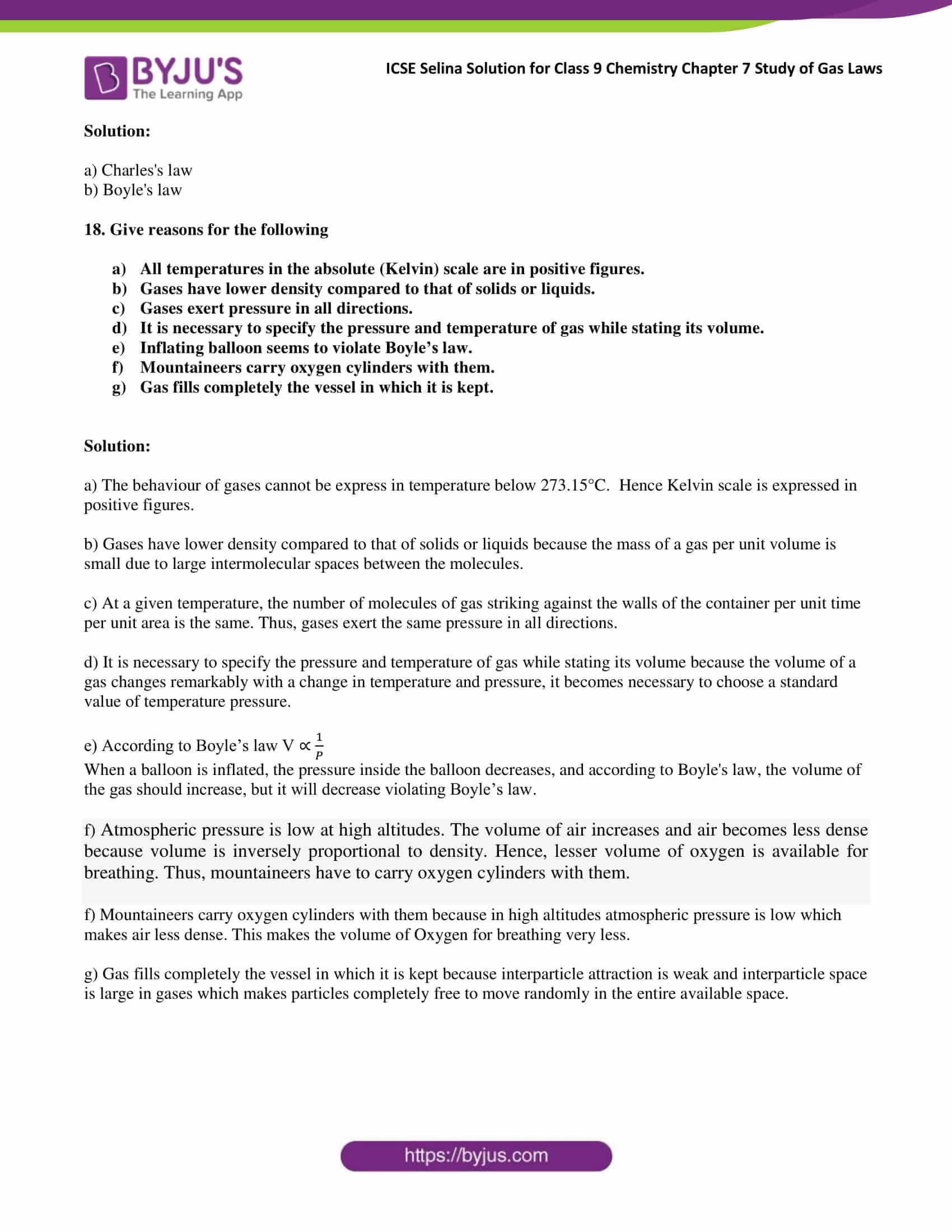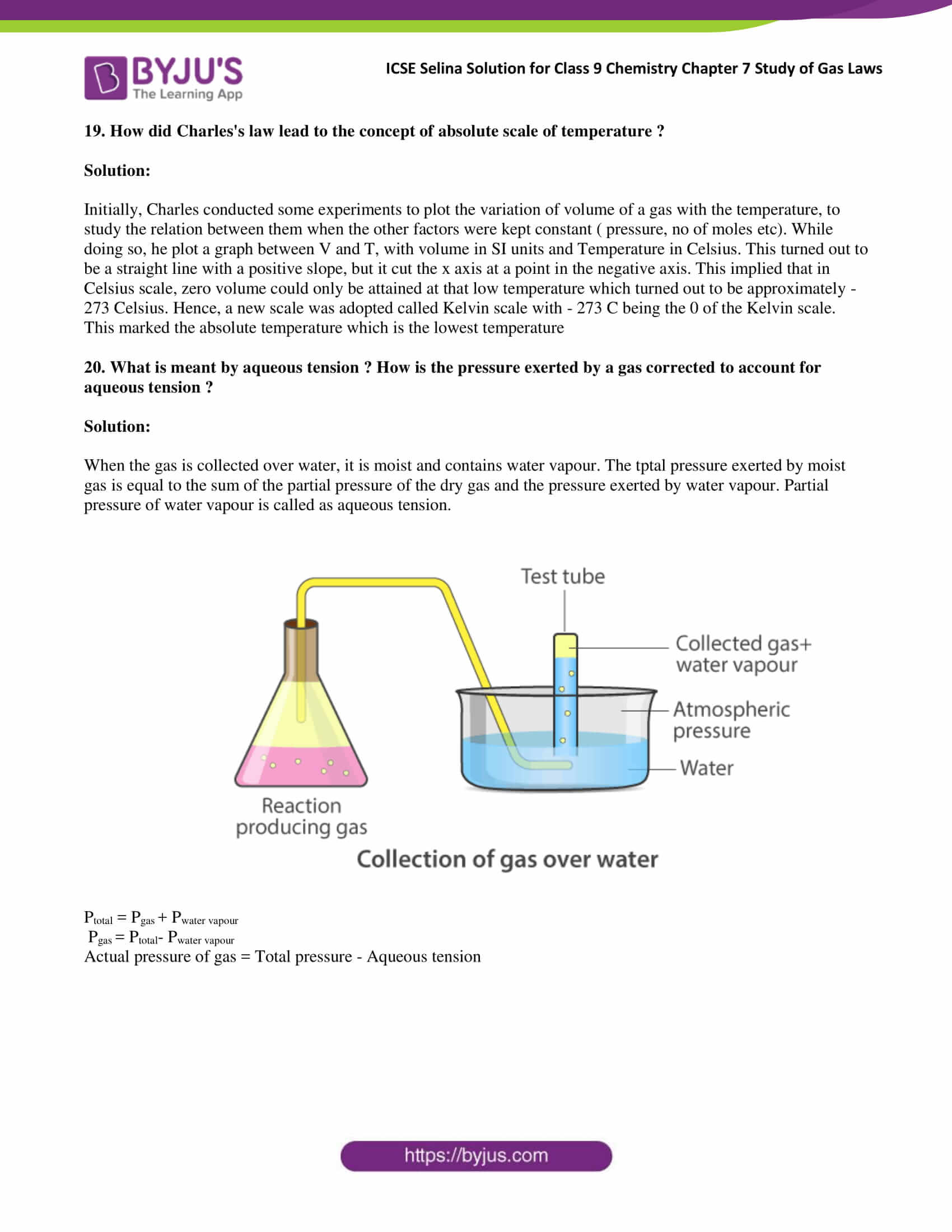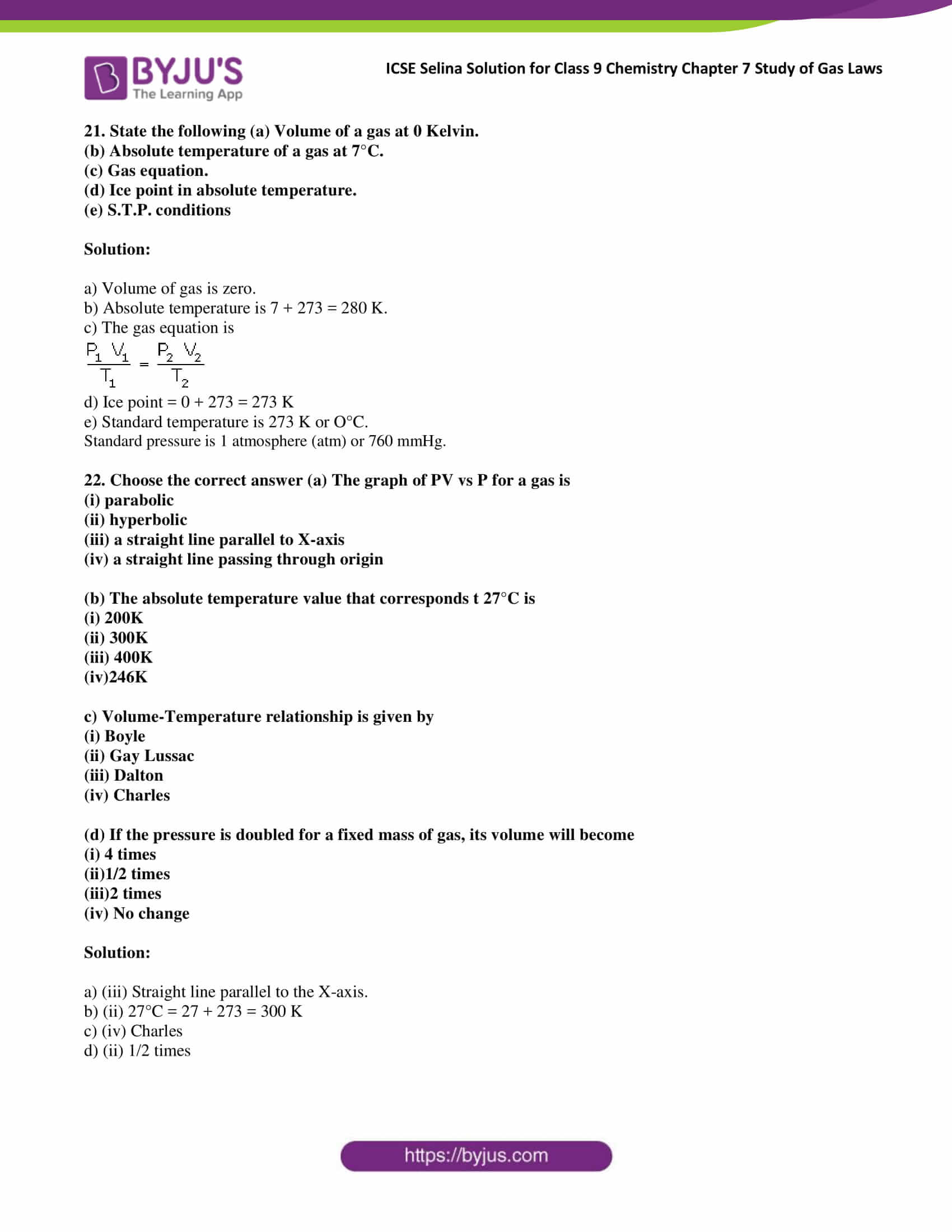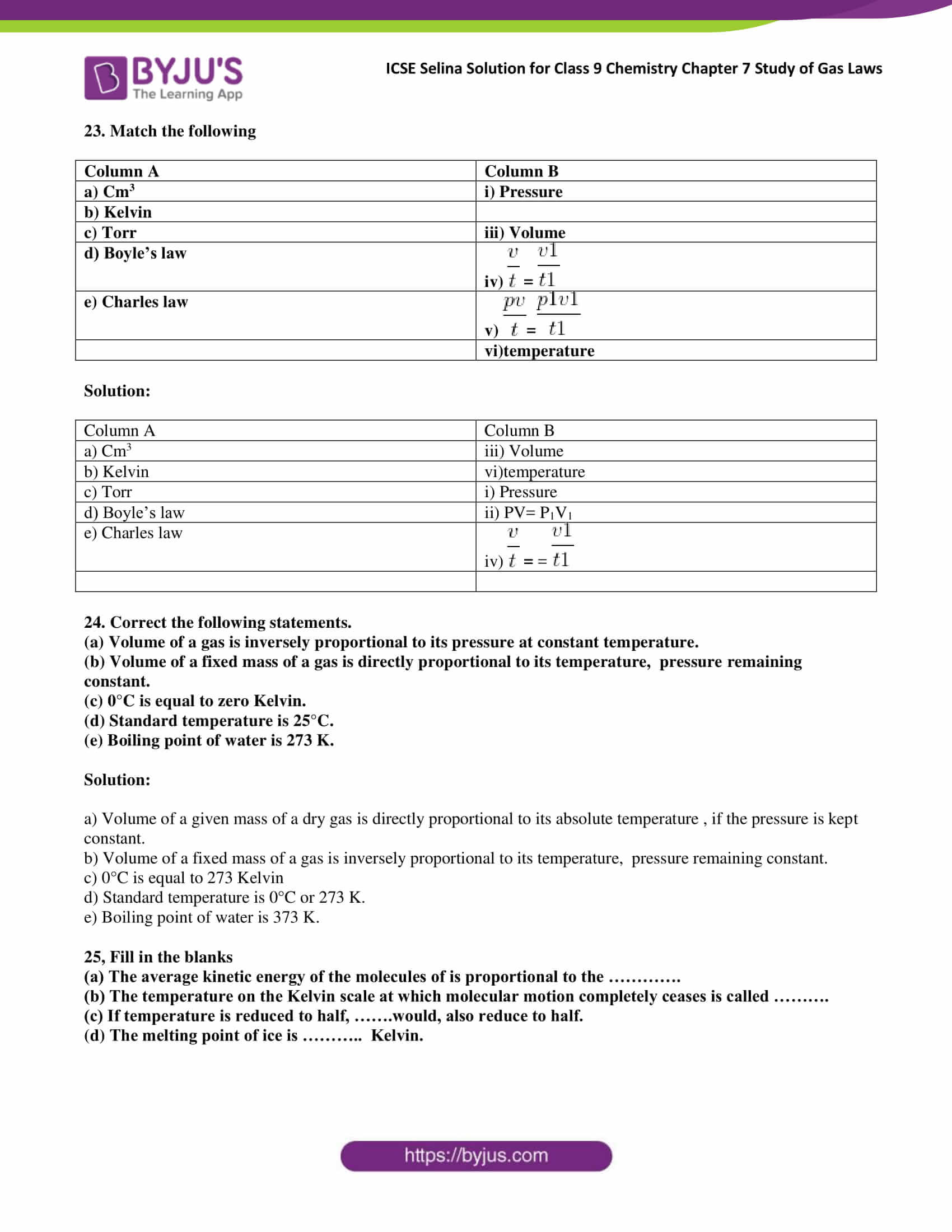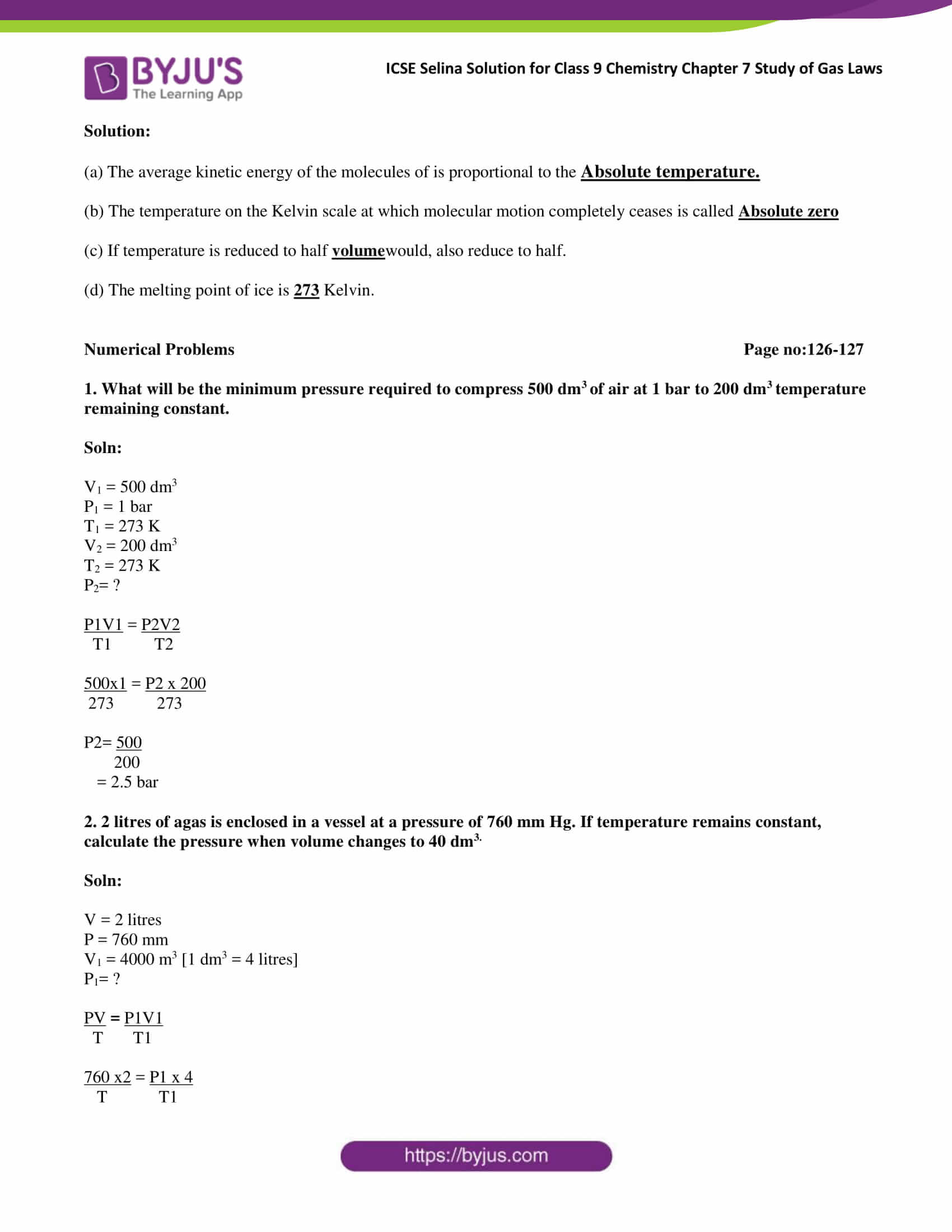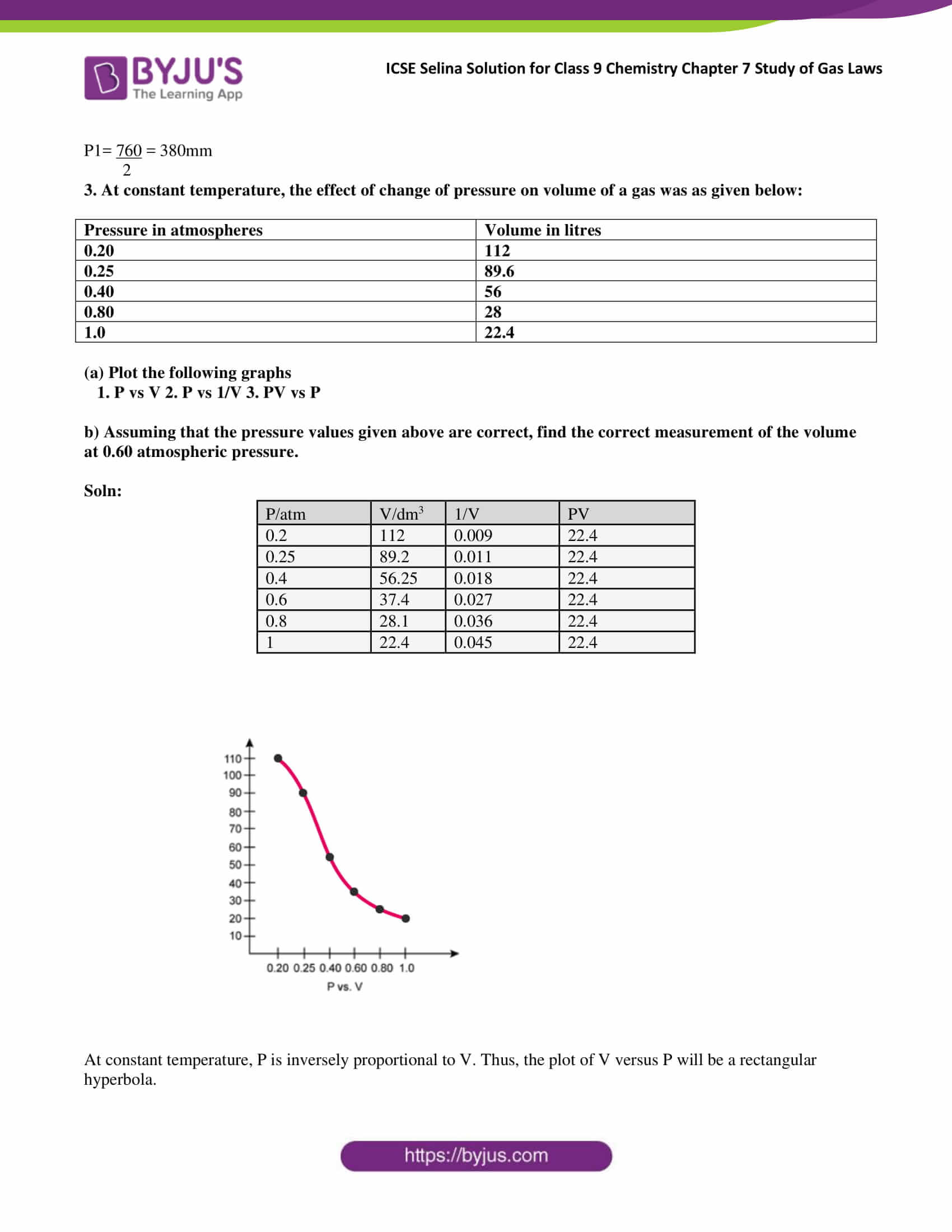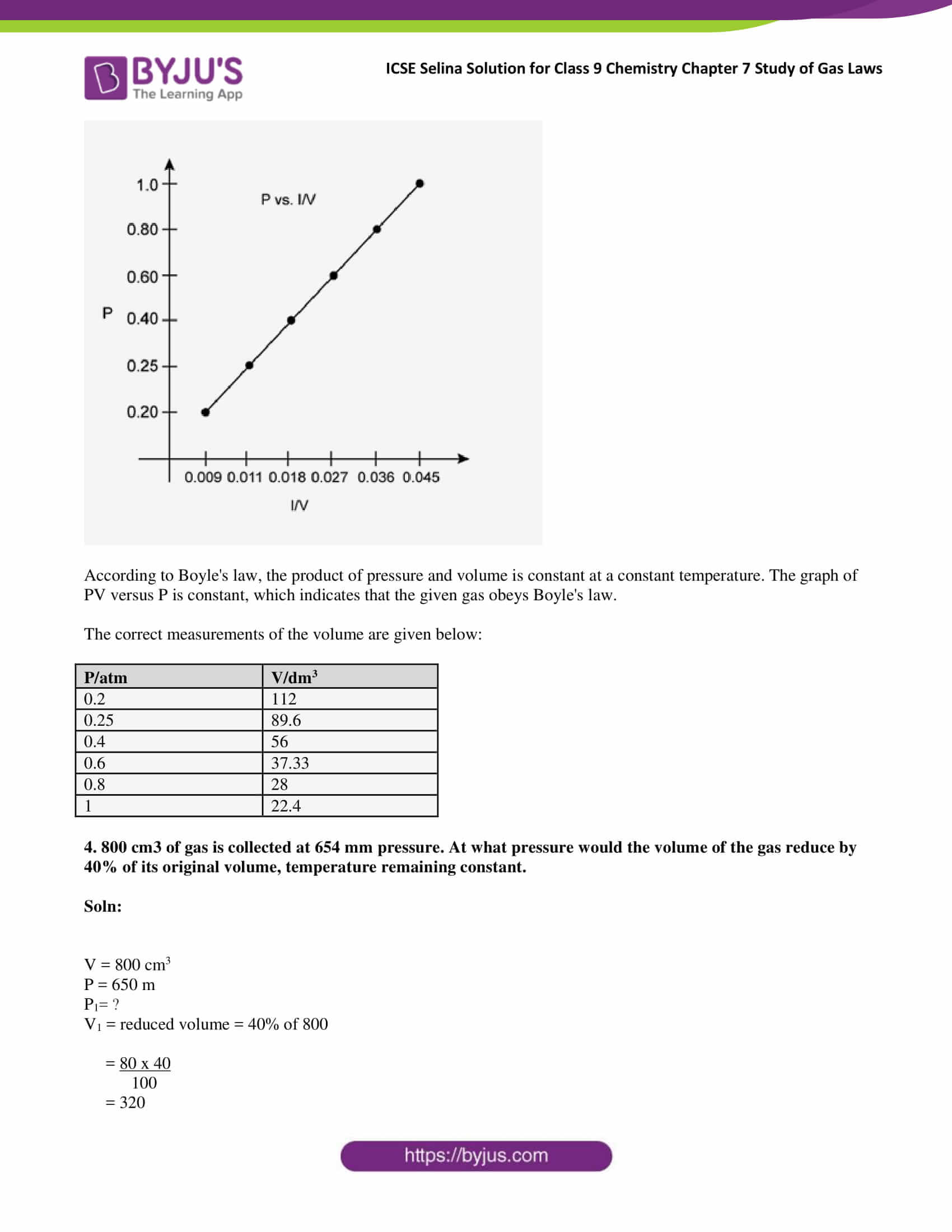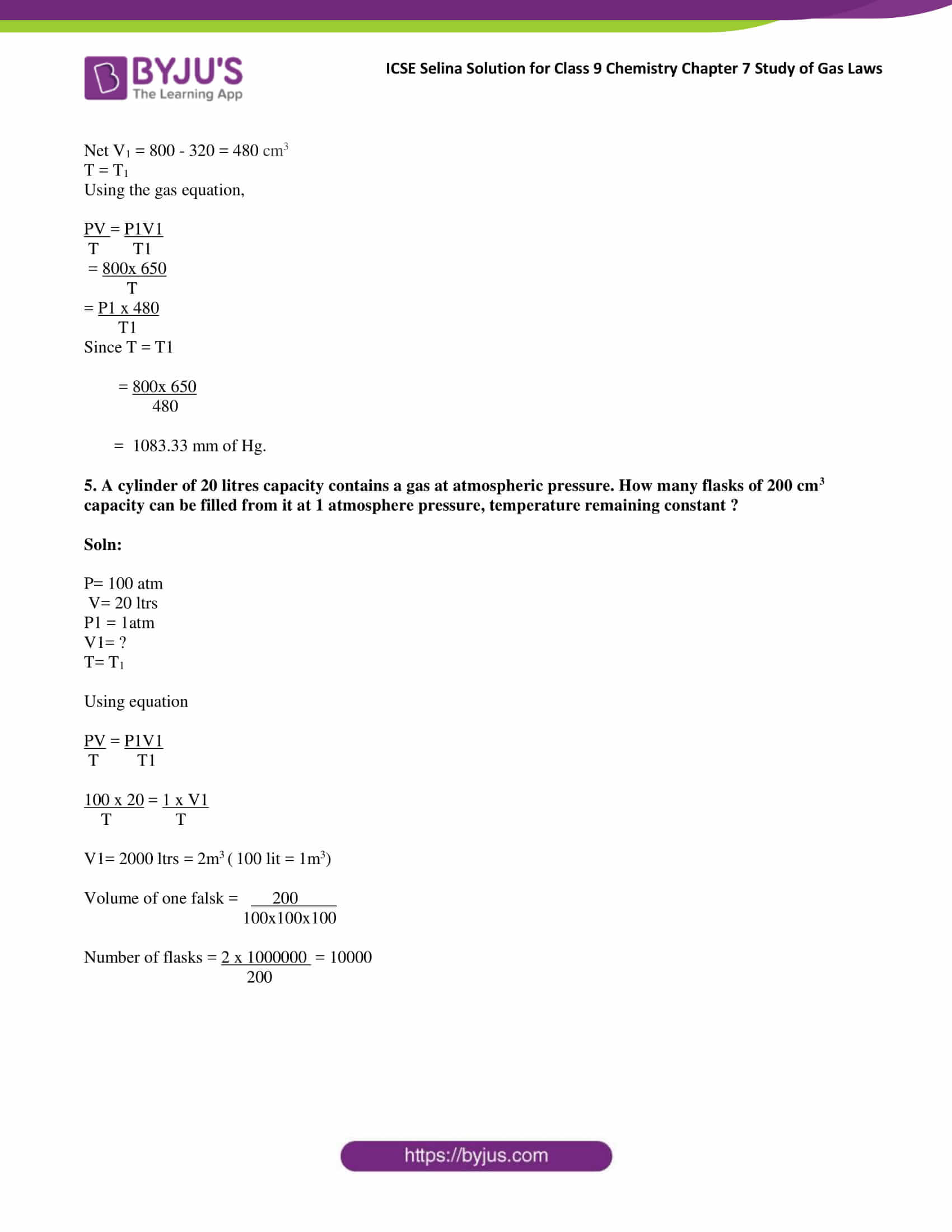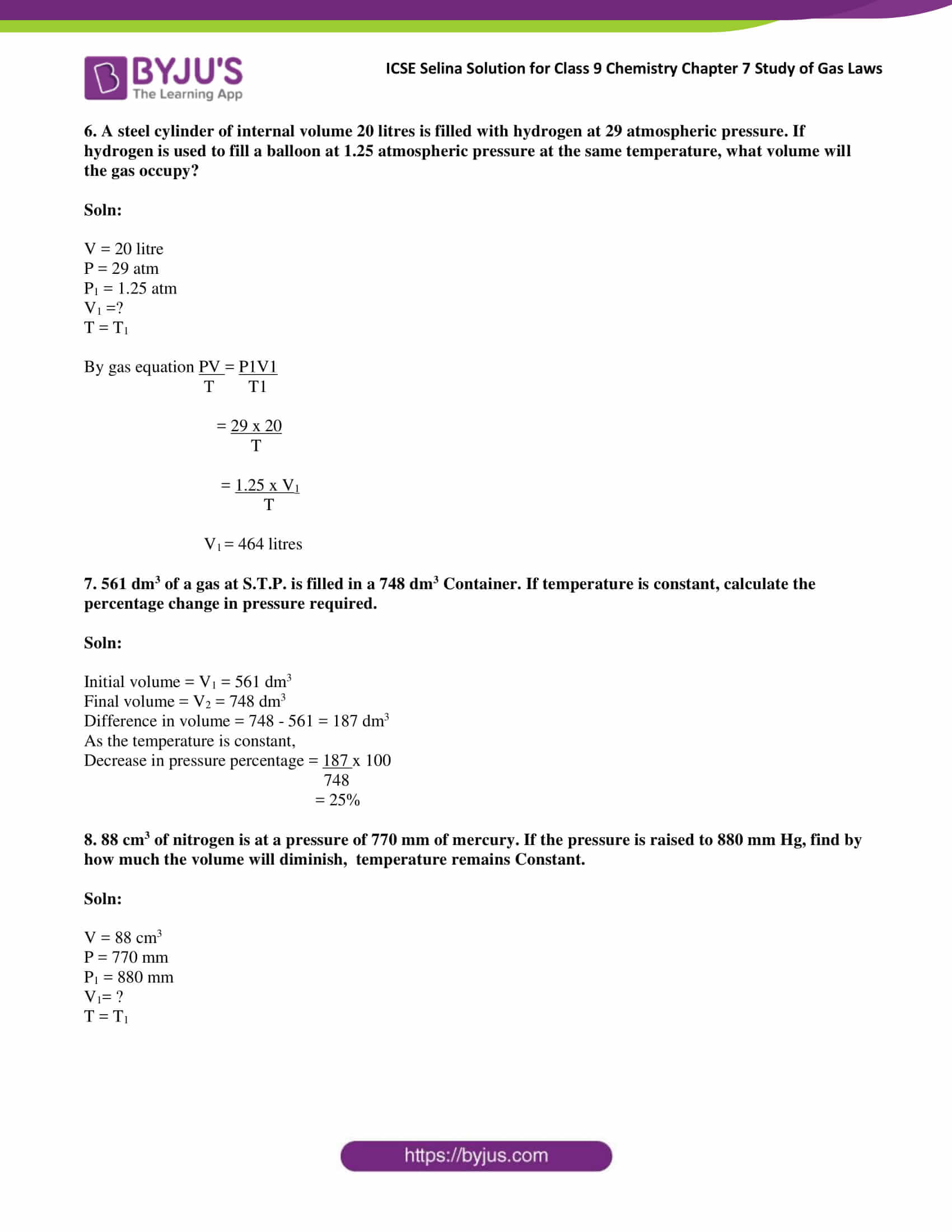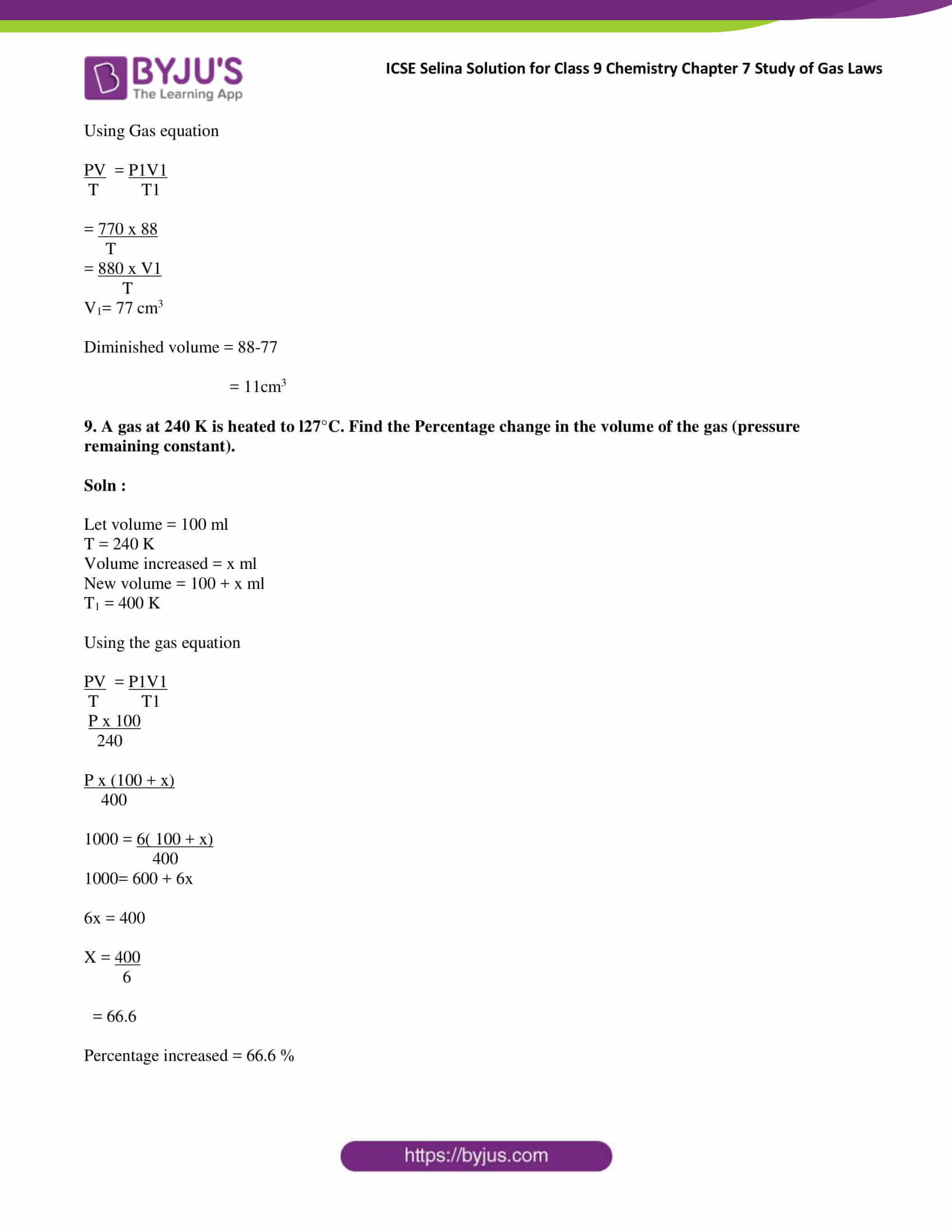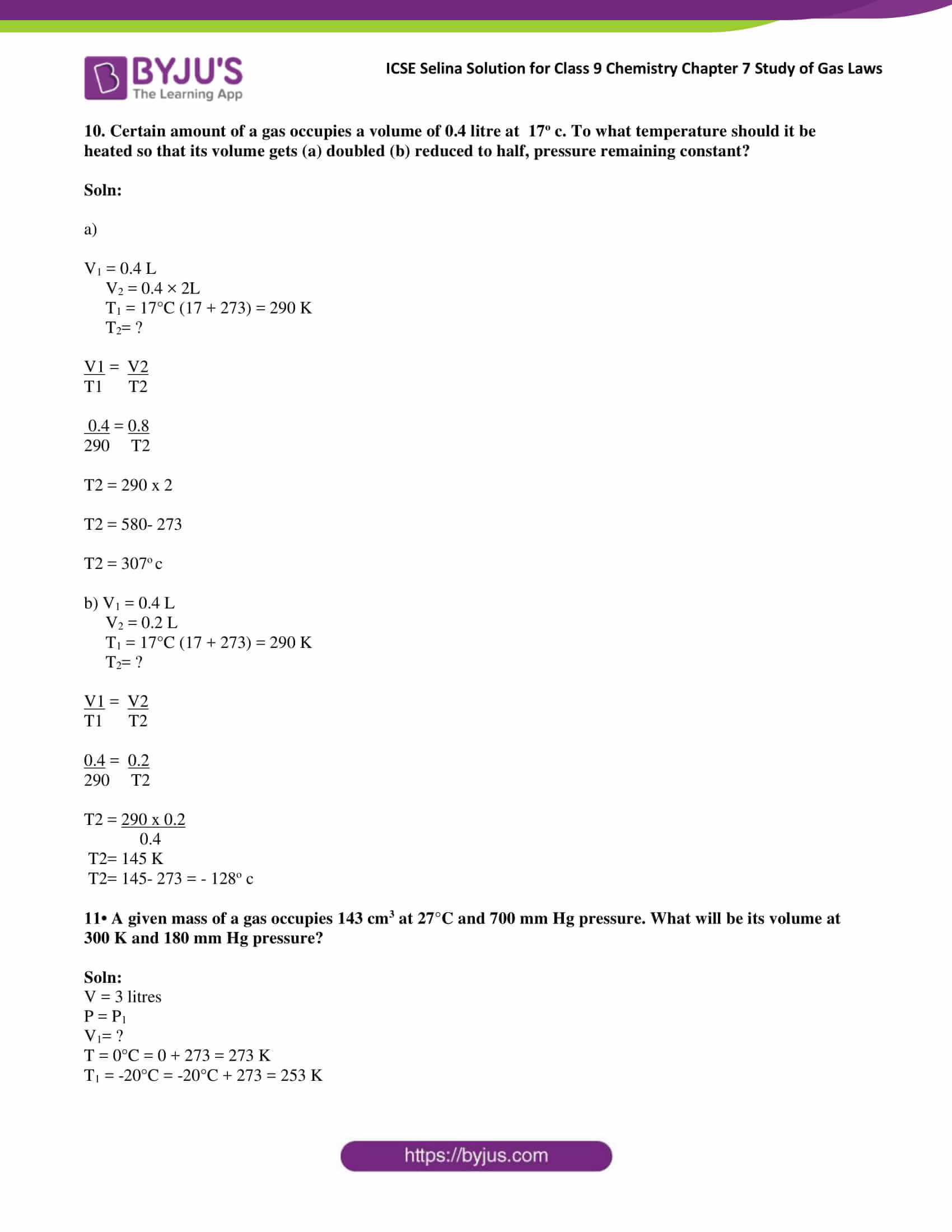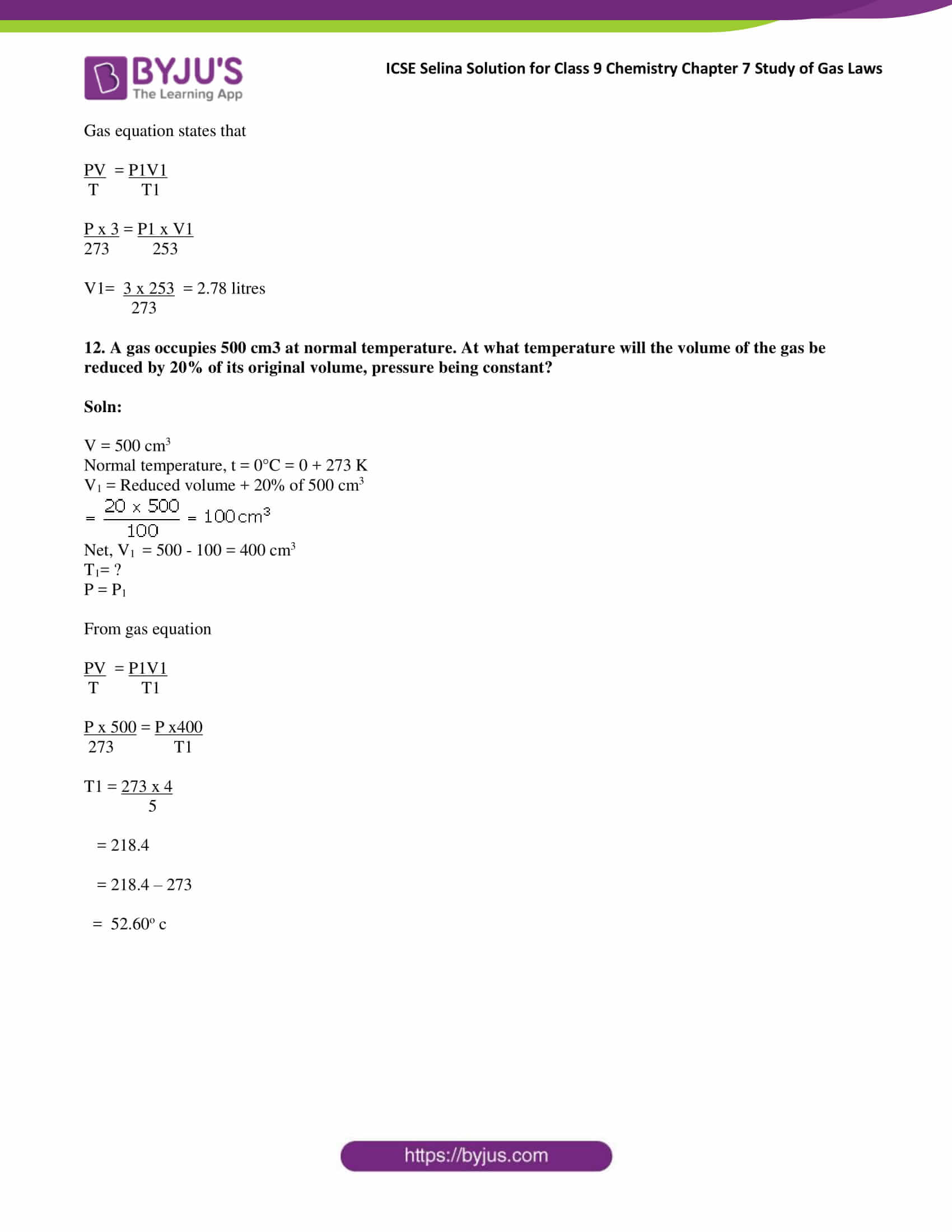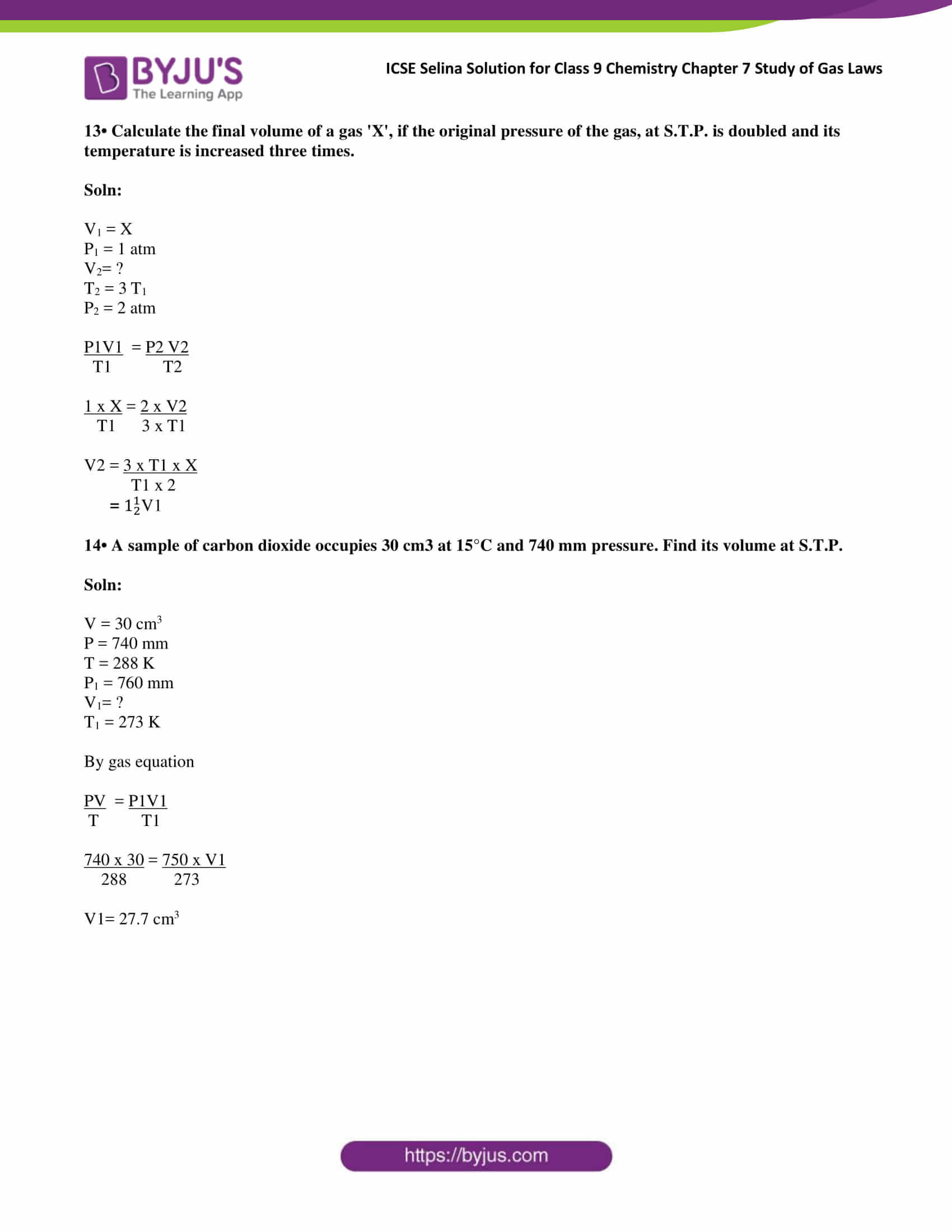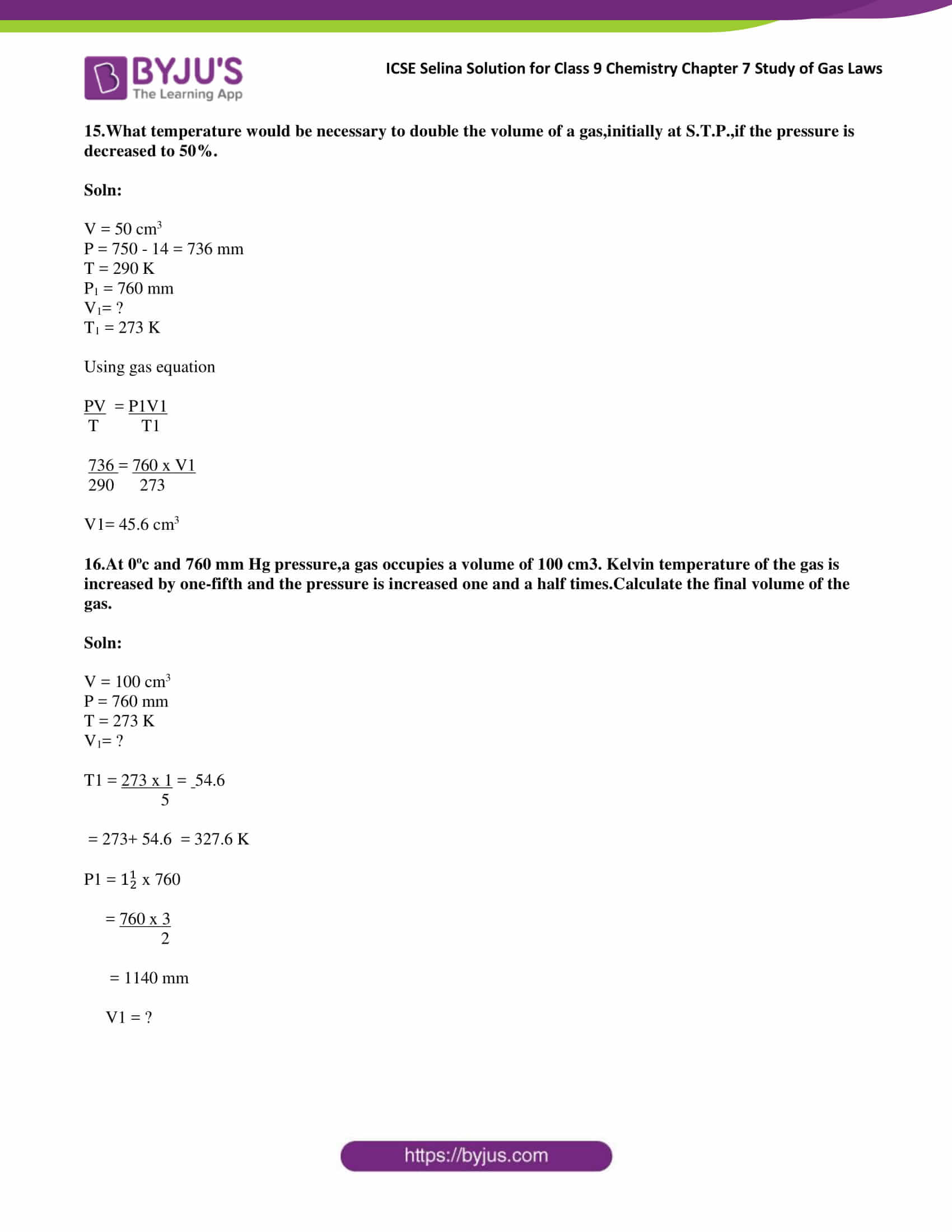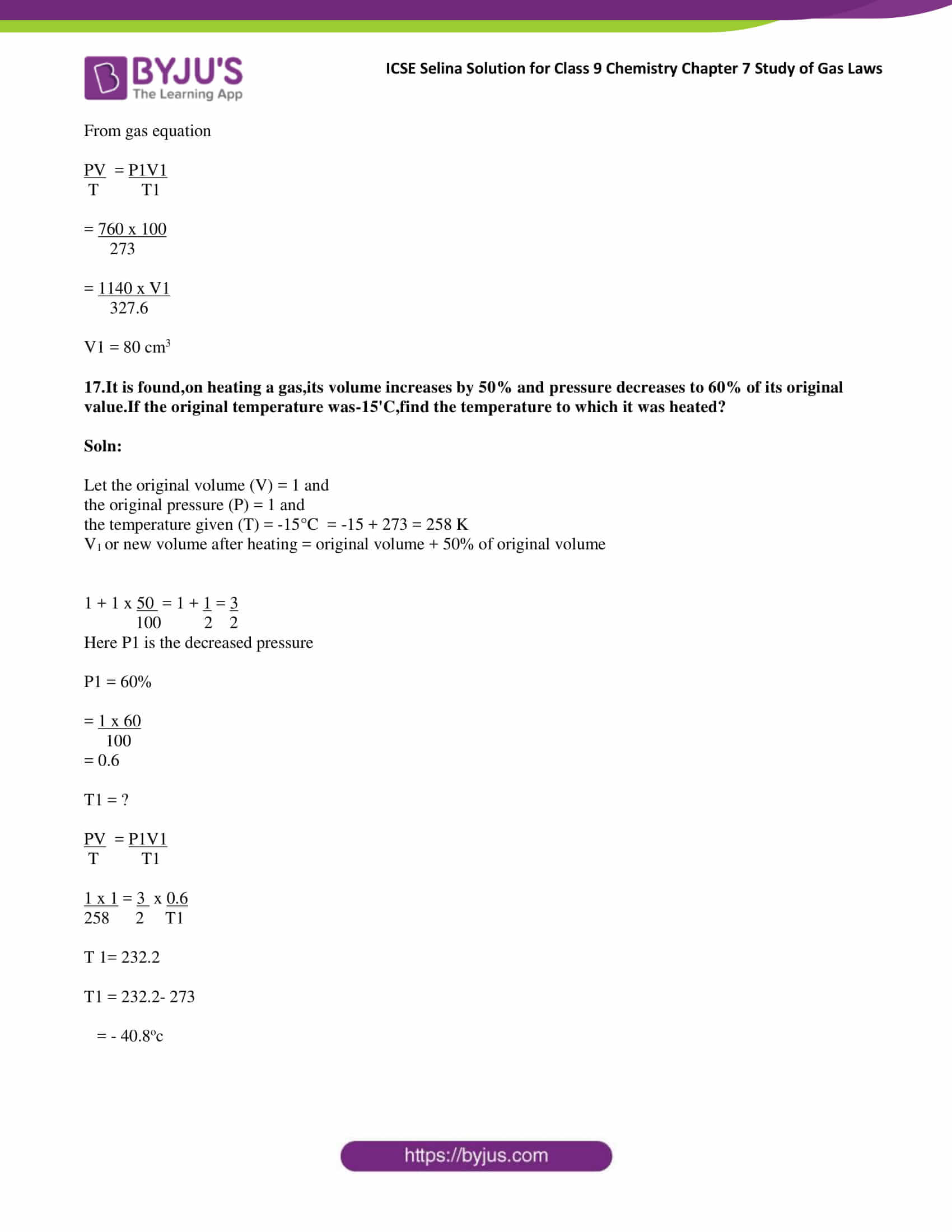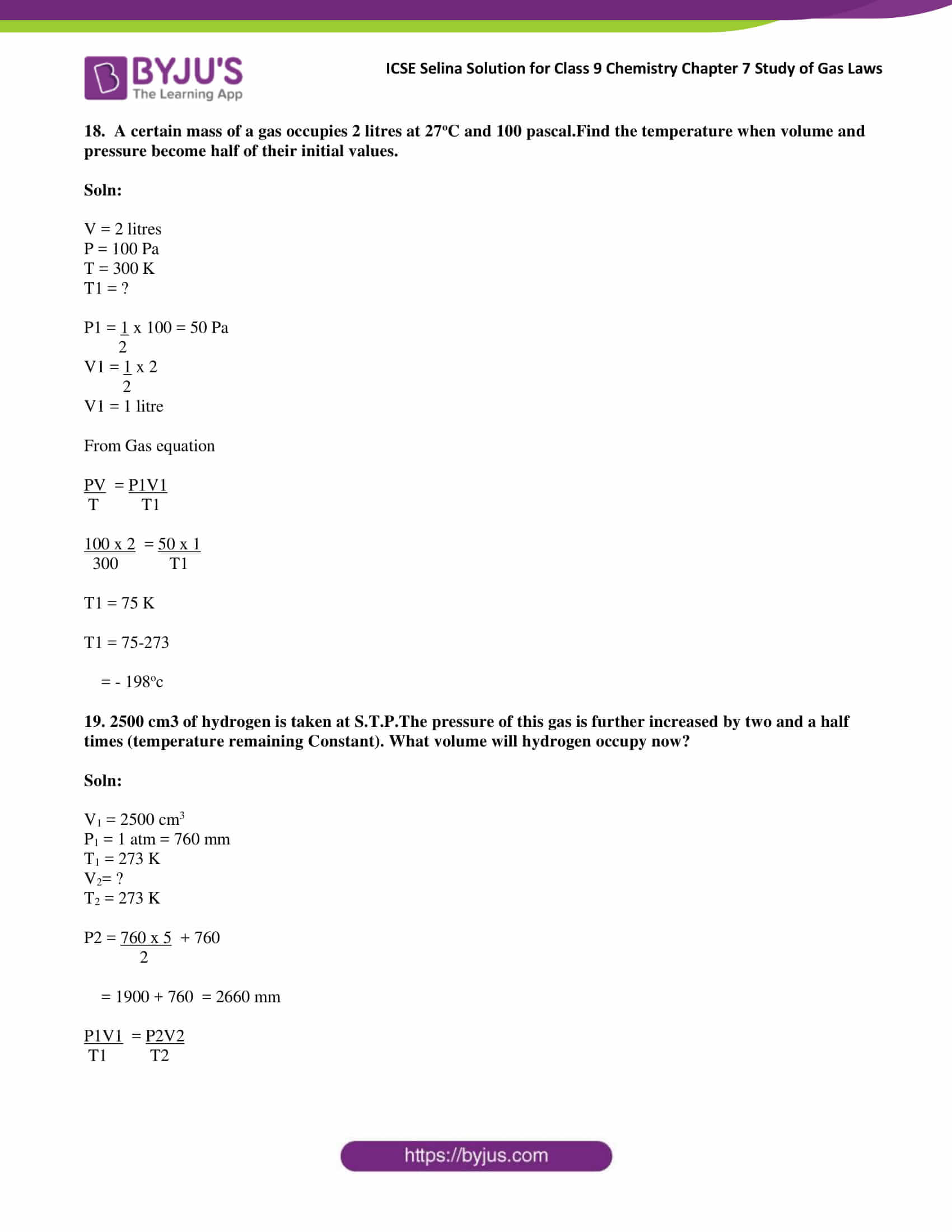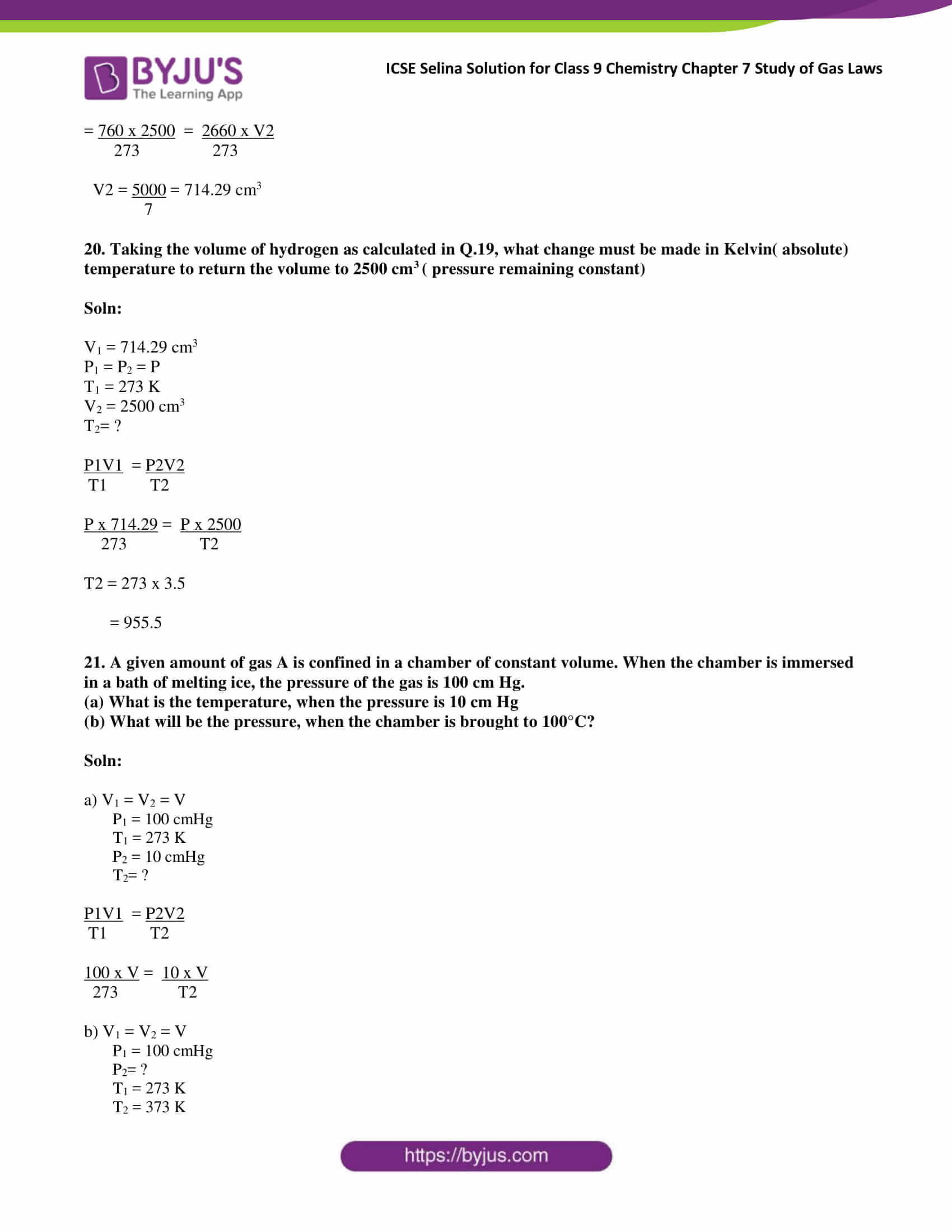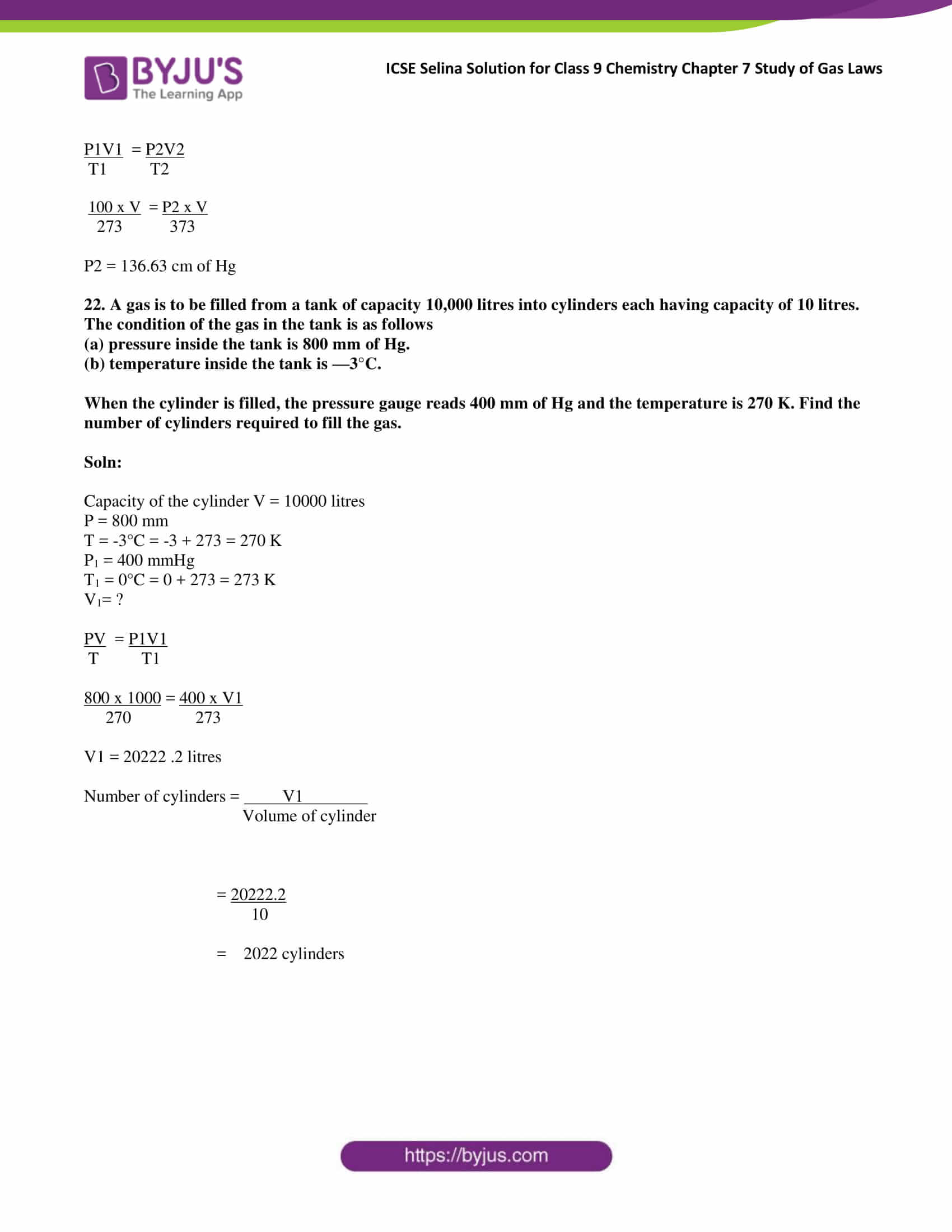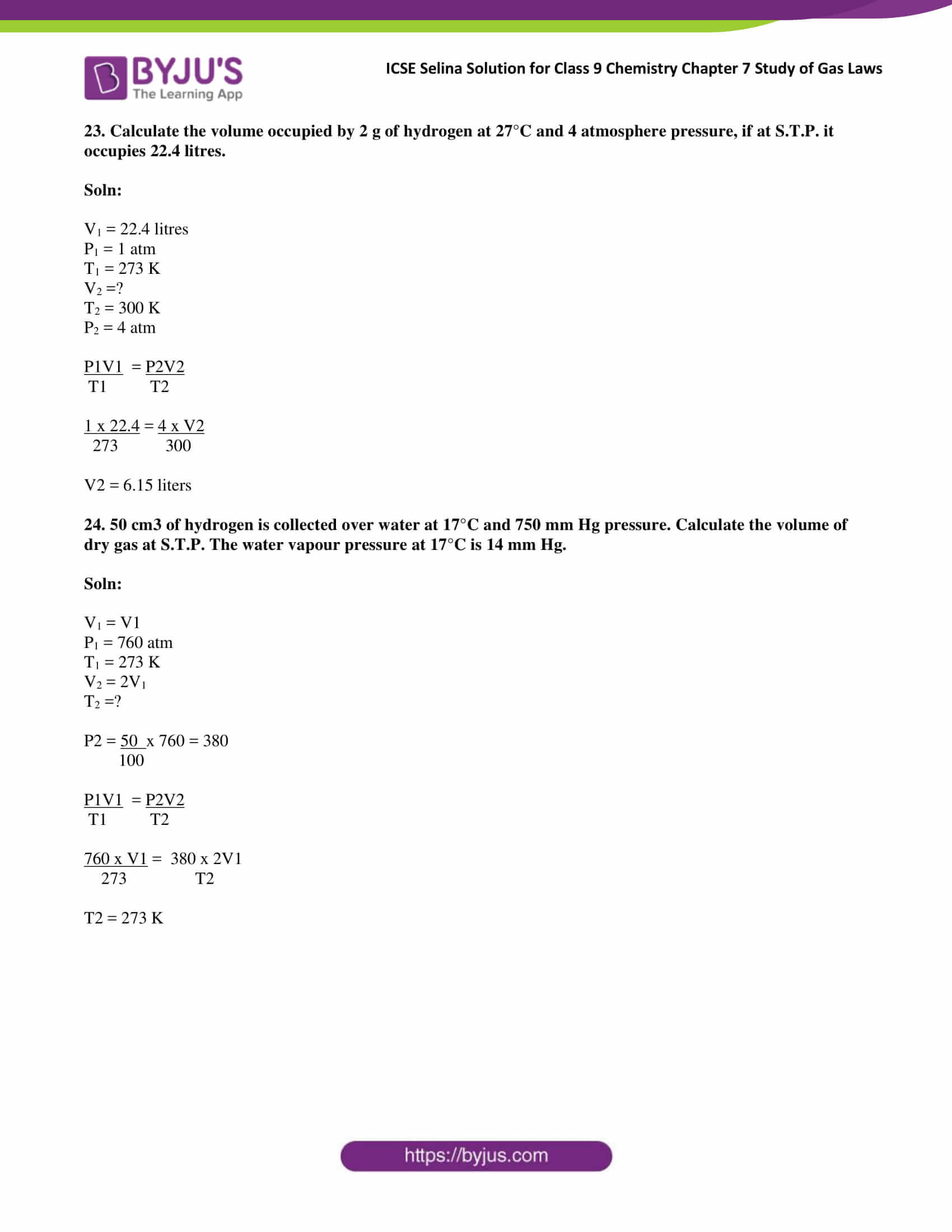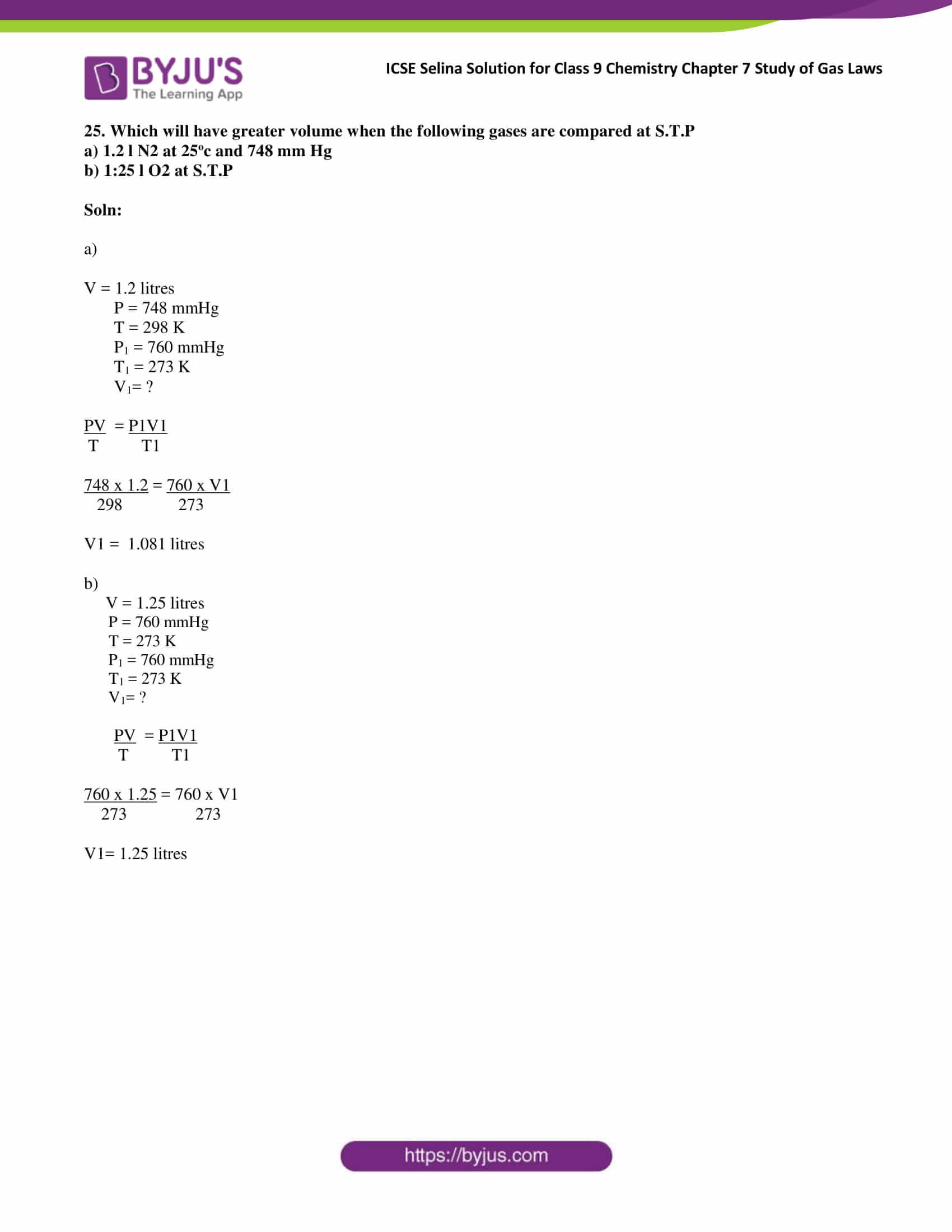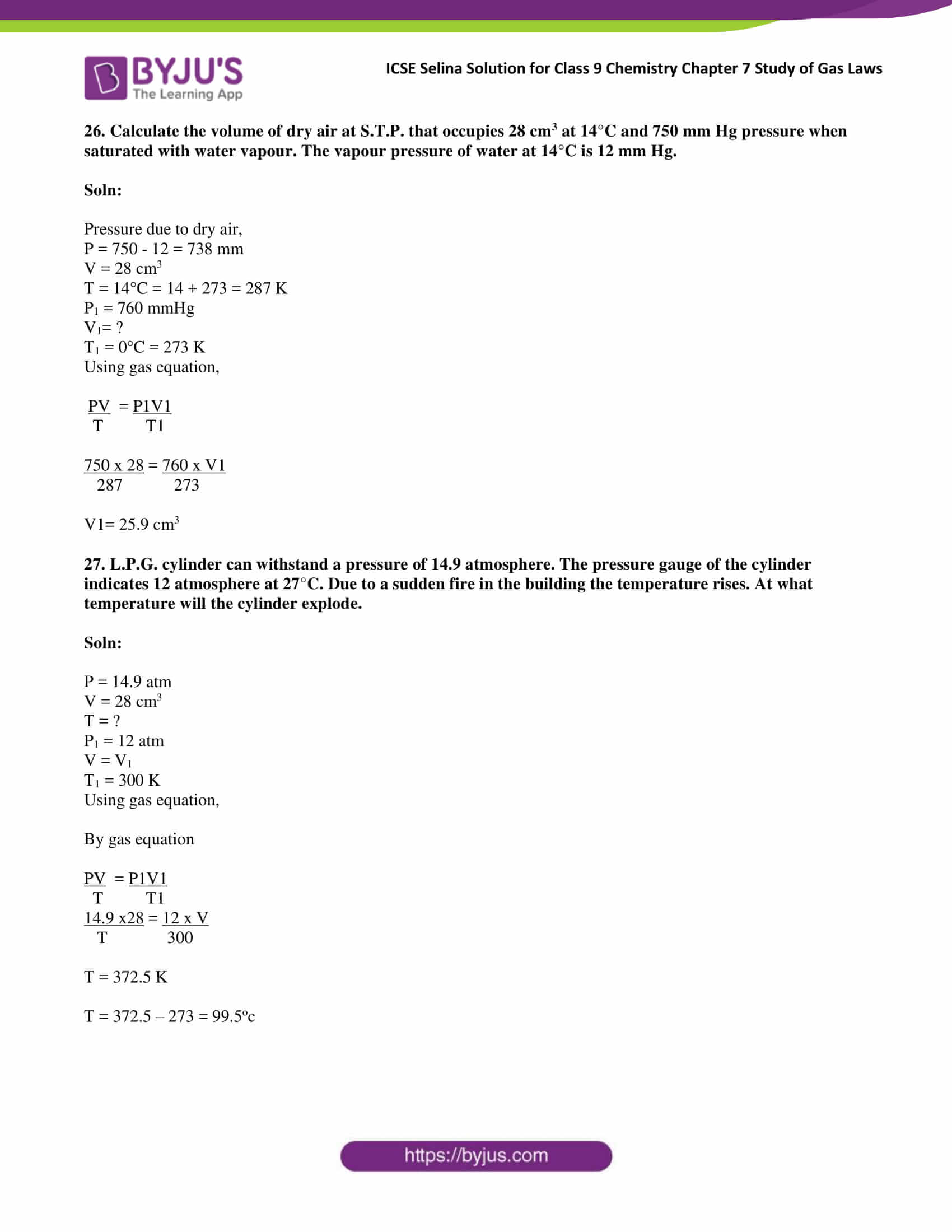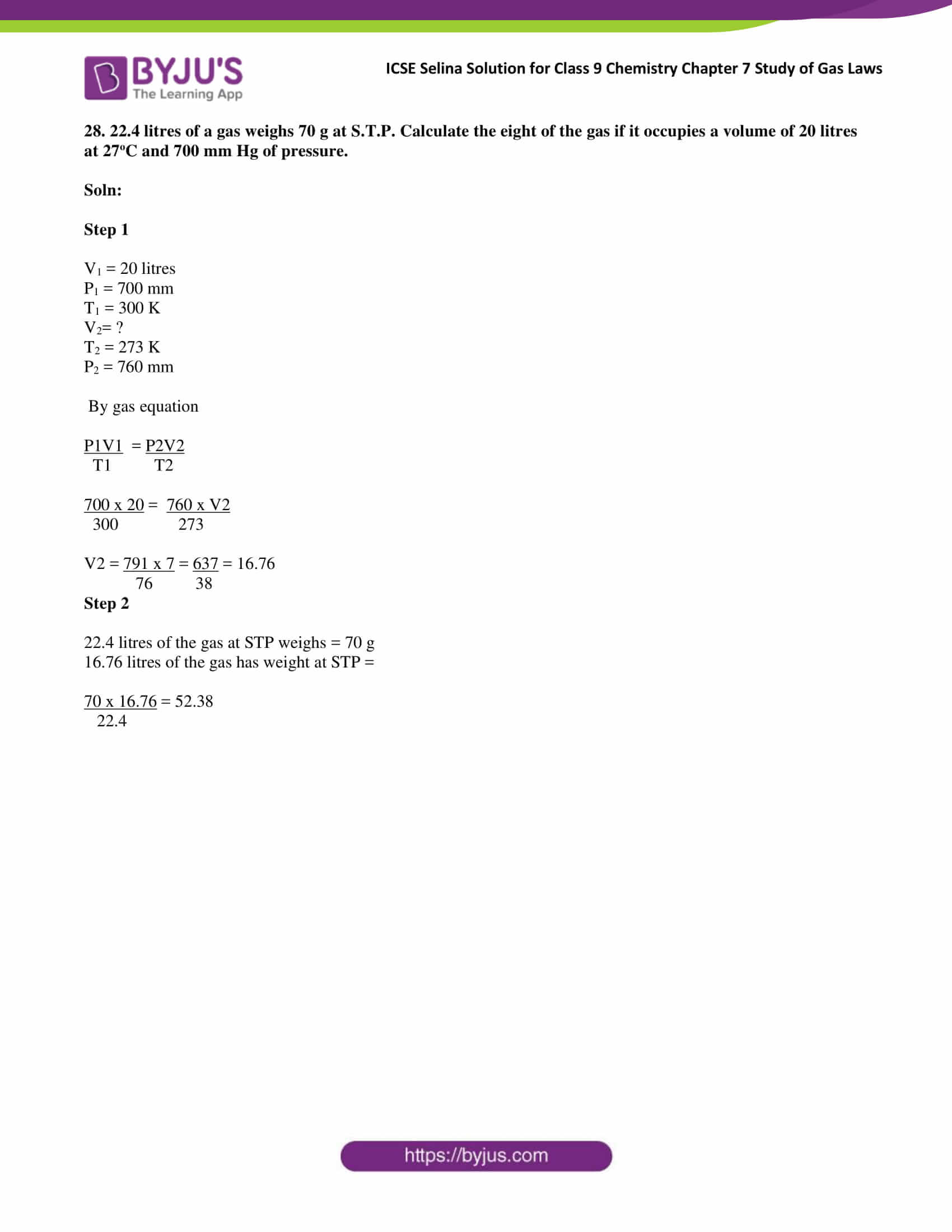### Access Answers of Selina publication ICSE Class 9 Chemistry Chapter 7 Study of Gas Laws

Exercise:7

1. What do you understand by gas?

Solution:

Gas is a physical state of matter in which interparticle attraction is weak and interparticle space is so large. Because of this gas particles become completely free to move randomly in the entire available space.

2. Give the assumptions of the kinetic molecular theory

Solution:

i) Gases are made of tiny particles which move in all possible directions at all possible speeds. The size of the molecules is small as compared to the volume of the occupied gas.

ii) There is no force of attraction between gas particles or between the particles and the walls of the container. So, the particles are free to move in the entire space available to them.

iii) The moving particles of gas collide with each other and with the walls of the container. Because of these collisions, gas molecules exert pressure. Gases exert the same pressure in all directions.

iv) There is a large interparticle space between gas molecules, and this accounts for high compressibility of gases.

v) The volume of a gas increases with a decrease in pressure and increase in temperature.

vi) Gases have a low density as they have large intermolecular spaces between their molecules.

vii) Gases have a natural tendency to mix with one and other because of large intermolecular spaces. So, two gases when mixed form a homogeneous gaseous mixture.

viii) The intermolecular space of a gas is reduced because of cooling. Molecules come closer resulting in liquefaction of the gas.

3. During the practical in the lab when hydrogen sulphide gas having offensive odour is prepared for some test, we can smell the gas even 50 metres away. Explain the phenomenon.

Solution:

We can smell the gas even 50 meters away because of the diffusion of gas molecules allow thorough mixing of the gas.

4. What is diffusion ? Give an example to illustrate

Solution:

Diffusion is a process of mixing of two substances kept in contact. Diffusion is due to molecular motion.

If a jar of chlorine is opened in a large room, the odorous gas mixes with air and spreads to every part of the room. Although chlorine is heavier than air, it does not remain at the floor level but spreads throughout the room.

5. How is molecular motion related to temperature?

Solution:

Molecular motion is directly proportional to temperature. Hence it affects the kinetic energy of the molecule.

6. State (i) the three variables for gas laws ii) S.I units of these variables.

Solution:

i) Three variables for gas laws: Volume (V), Pressure (P), Temperature (T)

ii) SI units of these variables:

Volume: Cubic metre (m3)

Pressure: Pascal (Pa)

Temperature: Kelvin (K)

7. (a) State Boyle’s Law.

(b) Give its

(i) mathematical expression,

(ii) graphical representation and

(iii) significance.

Solution:

a) Boyle’s law states that the volume of a given mass of a dry gas is inversely proportional to its pressure at a constant temperature.

b)

i. Mathematical representation:

According to Boyle’s Law,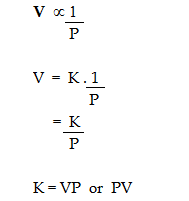where K is the constant of proportionality.

If V’ and P’ are some other volume and pressure of the gas at the same temperature, then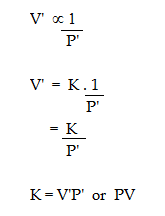ii. Graphical representation of Boyle’s Law: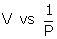1 : Variation in volume (V) plotted against (1/P) at a constant temperature: A straight line passing through the origin is obtained.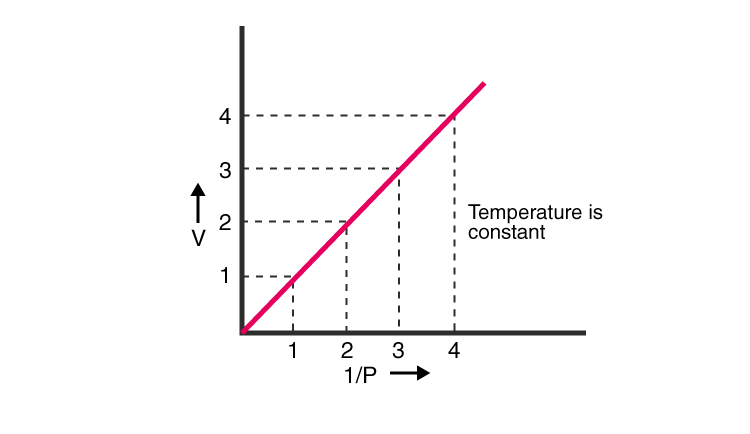2. V vs P: Variation in volume (V) plotted against pressure (P) at a constant temperature: A hyperbolic curve in the first quadrant is obtained.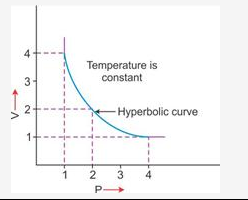3. PV vs P: Variation in PV plotted against pressure (P) at a constant temperature: A straight line parallel to the X-axis is obtained.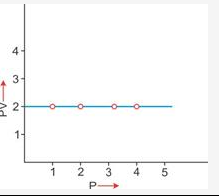iii) Significance of Boyle’s Law

On increasing pressure. volume decreases. The gas becomes denser. Thus at a constant temperature, the density of a gas is directly proportional to its pressure.

Atmospheric pressure is low at high altitudes, so air is less dense. Hence, a lesser quantity of oxygen is available for breathing. This is the reason why mountaineers have to carry oxygen cylinders with them.

8. Explain Boyle’s law on the basis of the kinetic theory of matter.

Solution:

The kinetic theory states that the number of molecules present in a given mass and the average possessed by the particles is constant.

If the volume of a given mass of dry gas is reduced to half its original volume, the same number of particles will have half the space to move. As a result, the number of molecules striking at a unit area of the walls of the container at a given time will get doubled. Conversely, if the volume of a given mass of a gas is doubled at a constant temperature, the same number of molecules will have double the space to move about. Consequently, the number of molecules striking at a unit area of the walls of the container at a given time will become one half of the original value. Thus, the pressure of the gas will be reduced to half of its original pressure. Hence it is observed that if pressure increases, the volume of a given mass of gas decreases at a constant temperature.

9 The molecular theory states that the pressure exerted by a gas in closed vessel results from the gas molecules striking’ against the walls of the vessel. How will the pressure change if :

(a) the temperature is doubled keeping the volume constant

(b) the volume is made half of its original value keeping the temperature constant?

Solution:

a) Pressure will double.

b) Pressure remains the same.

10.

1. State Charles law
2. Give its

(i) Graphical representation,

(ii) mathematical expression and

(iii) Significance.

Solution:

a)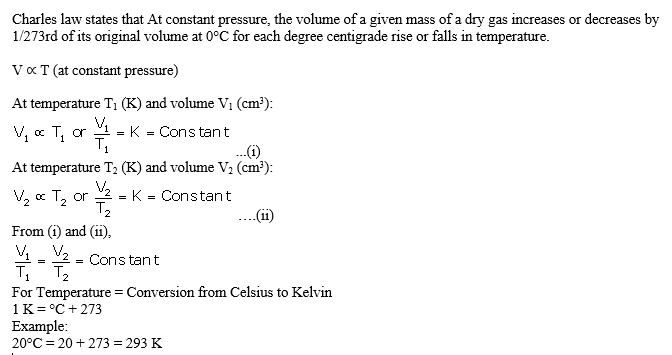b)

i) Graphical representation of Charles’s law

T vs V: The relationship between the volume and the temperature of a gas can be plotted on a graph. A straight line is obtained.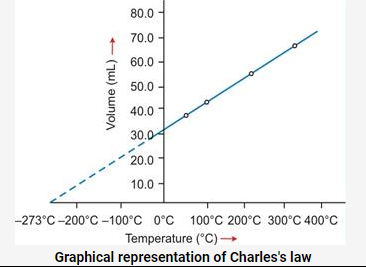ii)

V αT

Since V and T vary directly, we can equate them by making use of a constant k.

VT=constant=k

The value of k depends on the pressure of the gas, the amount of the gas and also on the unit of the volume.

VT = k ———– (I)

Let V1 and T1 be the initial volume and temperature of an ideal gas. We can write the equation I as:

V1T1=k ———– (II)

Let’s change the temperature of the gas to T2. Consequently, its volume changes to V2. So we can write,

V2T2=k ———– (III)

Equating equations (II) and (III),

V1T1=V2T2=k

Hence, we can generalize the formula and write it as:

(V1)(T1)=(V2)(T2)

Or

V1T2=V2T1

iii)

Significance of Charles’s Law: The volume of a given mass of a gas is directly proportional to its temperature; hence, the density decreases with temperature. This is the reason that

(a) Hot air is filled in balloons used for meteorological purposes. (b) Cable wires contract in winters and expand in summers.

11. Explain Charles’s law on the basis of the kinetic theory of matter.

Solution:

According to the kinetic theory of matter, the average kinetic energy of gas molecules is directly proportional to the absolute temperature. Thus, when the temperature of a gas is increased, the molecules would move faster and the molecules will strike the unit area of the walls of the container more frequently and vigorously. If the pressure is kept constant, the volume increases proportionately. Hence, at constant pressure, the volume of a given mass of a gas is directly proportional to the temperature (Charles’s law).

12. Define absolute zero and absolute scale of temperature. Write about the relationship between °C and K.

Solution:

Absolute zero is – 273 °C. The value on the Celsius scale can be converted to the Kelvin scale by adding 273 to it.

Example: 20°C = 20 + 273 = 293 K .

13. (a) What is the need for the Kelvin scale of temperature?

(b) What is the boiling point of water on the Kelvin scale? Convert it into a centigrade scale.

Solution:

The behaviour of gases cannot be express in temperature below 273.15°C. Hence there was need of Kelvin scale to express behaviour.

The boiling point of water on the Kelvin scale is 373 K.

In Centigare 372-273= 100 °C

14. (a) Define S.T.P.

(b) Why is it necessary to compare gases at S.T.P.?

Solution:

The temperature of 0°C or 273 K, the pressure of 76mm Hg or 1 atm is considered as standard temperature and pressure.

It is necessary to compare gases at STP because the volume of a gas changes remarkably with a change in temperature.

15. Write the value of

a) Standard temperature in

i) °C ii) K

b) Standard pressure in

i) atm ii) mmHg iii) cm Hg iv) torr

Solution:

Standard temperature i) 0°C ii)273 K

b) Standard pressure i) 1 atmospheric pressure (atm)  ii) 760 mmHg iii) 76 cm of Hg iv) 101325760 pascals

16. What is the relationship between the Celsius and the Kelvin scales of temperature?

Solution:

Temperature on the Kelvin scale (K)

= 273 + temperature on the Celsius scale

Or K = 273 + °C

(i) 273°C in Kelvin

t°C = t K – 273

273°C = t K – 273

t K = 273 + 273 = 546 K

∴ 273°C = 546 K

(ii) 293 K in °C

t°C = 293 – 273

t°C = 20°C

∴ 293 K = 20°C

17. State the laws which are represented by the following graphs.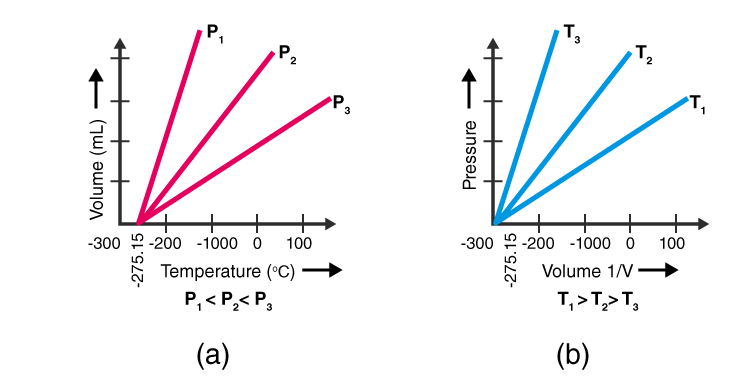Solution:

a) Charles’s law

b) Boyle’s law

18. Give reasons for the following

1. All temperatures in the absolute (Kelvin) scale are in positive figures.
2. Gases have a lower density compared to that of solids or liquids.
3. Gases exert pressure in all directions.
4. It is necessary to specify the pressure and temperature of gas while stating its volume.
5. The inflating balloon seems to violate Boyle’s law.
6. Mountaineers carry oxygen cylinders with them.
7. Gas fills completely the vessel in which it is kept.

Solution:

a) The behaviour of gases cannot be express in temperature below 273.15°C. Hence Kelvin scale is expressed in positive figures.

b) Gases have lower density compared to that of solids or liquids because the mass of a gas per unit volume is small due to large intermolecular spaces between the molecules.

c) At a given temperature, the number of molecules of gas striking against the walls of the container per unit time per unit area is the same. Thus, gases exert the same pressure in all directions.

d) It is necessary to specify the pressure and temperature of gas while stating its volume because the volume of a gas changes remarkably with a change in temperature and pressure, it becomes necessary to choose a standard value of temperature pressure.

e) According to Boyle’s law V ∝

When a balloon is inflated, the pressure inside the balloon decreases, and according to Boyle’s law, the volume of the gas should increase, but it will decrease violating Boyle’s law.

f) Atmospheric pressure is low at high altitudes. The volume of air increases and the air becomes less dense because the volume is inversely proportional to density. Hence, a lesser volume of oxygen is available for breathing. Thus, mountaineers have to carry oxygen cylinders with them.

f) Mountaineers carry oxygen cylinders with them because in high altitudes atmospheric pressure is low which makes air less dense. This makes the volume of Oxygen for breathing very less.

g) Gas fills completely the vessel in which it is kept because interparticle attraction is weak and interparticle space is large in gases which makes particles completely free to move randomly in the entire available space.

19. How did Charles’s law lead to the concept of an absolute scale of temperature?

Solution:

Initially, Charles conducted some experiments to plot the variation of volume of a gas with the temperature, to study the relationship between them when the other factors were kept constant ( pressure, no of moles etc). While doing so, he plot a graph between V and T, with volume in SI units and Temperature in Celsius. This turned out to be a straight line with a positive slope, but it cut the x-axis at a point in the negative axis. This implied that in Celsius scale, zero volume could only be attained at that low temperature which turned out to be approximate – 273 Celsius. Hence, a new scale was adopted called the Kelvin scale with – 273 C being the 0 of the Kelvin scale. This marked the absolute temperature which is the lowest temperature

20. What is meant by aqueous tension? How is the pressure exerted by a gas corrected to account for aqueous tension?

Solution:

When the gas is collected over water, it is moist and contains water vapour. The total pressure exerted by moist gas is equal to the sum of the partial pressure of the dry gas and the pressure exerted by water vapour. The partial pressure of water vapour is called as aqueous tension.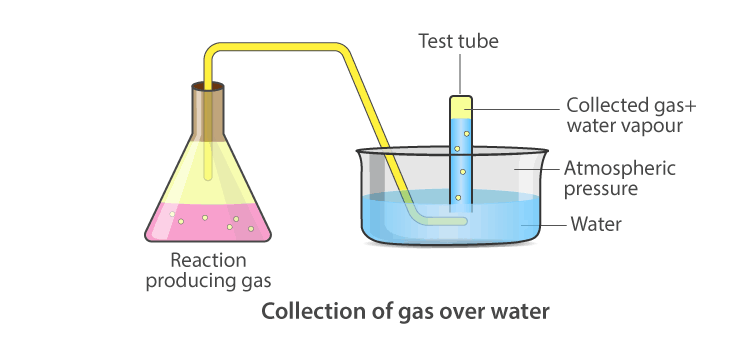Ptotal = Pgas + Pwater vapour

Pgas = Ptotal– Pwater vapour

Actual pressure of gas = Total pressure – Aqueous tension

21. State the following (a) Volume of a gas at 0 Kelvin.

(b) The absolute temperature of a gas at 7°C.

(c) Gas equation.

(d) Ice point in absolute temperature.

(e) S.T.P. conditions

Solution:

a) Volume of gas is zero.

b) Absolute temperature is 7 + 273 = 280 K.

c) The gas equation is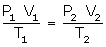d) Ice point = 0 + 273 = 273 K

e) Standard temperature is 273 K or O°C.

Standard pressure is 1 atmosphere (atm) or 760 mmHg.

22. Choose the correct answer (a) The graph of PV vs P for gas is

(i) parabolic

(ii) hyperbolic

(iii) a straight line parallel to X-axis

(iv) a straight line passing through the origin

(b) The absolute temperature value that corresponds t 27°C is

(i) 200K

(ii) 300K

(iii) 400K

(iv)246K

c) A volume-Temperature relationship is given by

(i) Boyle

(ii) Gay Lussac

(iii) Dalton

(iv) Charles

(d) If the pressure is doubled for a fixed mass of gas, its volume will become

(i) 4 times

(ii)1/2 times

(iii)2 times

(iv) No change

Solution:

a) (iii) Straight line parallel to the X-axis.

b) (ii) 27°C = 27 + 273 = 300 K

c) (iv) Charles

d) (ii) 1/2 times

23. Match the following

 Column A Column B a) Cm3 i) Pressure b) Kelvin c) Torr iii) Volume d) Boyle’s law iv)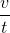=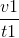e) Charles law v)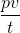=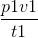vi)temperature

Solution:

 Column A Column B a) Cm3 iii) Volume b) Kelvin vi)temperature c) Torr i) Pressure d) Boyle’s law ii) PV= P1V1 e) Charles law iv)= =24. Correct the following statements.

(a) The volume of a gas is inversely proportional to its pressure at a constant temperature.

(b) The volume of a fixed mass of a gas is directly proportional to its temperature, pressure remaining constant.

(c) 0°C is equal to zero Kelvin.

(d) Standard temperature is 25°C.

(e) The boiling point of water is 273 K.

Solution:

a) The volume of a given mass of a dry gas is directly proportional to its absolute temperature if the pressure is kept constant.

b) The volume of a fixed mass of a gas is inversely proportional to its temperature, pressure remaining constant.

c) 0°C is equal to 273 Kelvin

d) Standard temperature is 0°C or 273 K.

e) The boiling point of water is 373 K.

25, Fill in the blanks

(a) The average kinetic energy of the molecules is proportional to the ………….

(b) The temperature on the Kelvin scale at which molecular motion completely ceases is called ……….

(c) If the temperature is reduced to half, …….would, also reduce to half.

(d) The melting point of ice is ……….. Kelvin.

Solution:

(a) The average kinetic energy of the molecules is proportional to the Absolute temperature.

(b) The temperature on the Kelvin scale at which molecular motion completely ceases is called Absolute zero

(c) If the temperature is reduced to the half volume would also reduce to half.

(d) The melting point of ice is 273 Kelvin.

## Important topics covered in ICSE Selina Solution for Class 9 Chemistry Chapter 7

• Behaviour and characteristic properties of Gases
• Molecular motion: Relationship of Temperature, Pressure and Volume
• Gas Laws
• Pressure and Volume Relationship in gases
• Temperature- Volume relationship in gases
• Absolute Zero
• Absolute or Kelvin Scale of Temperature
• Conversion of temperature from Celsius scale to Kelvin scale and vice-versa
• The Gas equation
• Standard temperature and pressure
• Effect of moisture or pressure

To get free access to ICSE syllabus books, previous year question papers, sample papers, important questions, ICSE marking scheme of all the classes and subjects, download BYJU’S learning App.

## Frequently Asked Questions on the Study of Gas laws

### What do you understand by gas?

Gas is a physical state of matter in which interparticle attraction is weak and interparticle space is so large. Because of this gas particles become completely free to move randomly in the entire available space.

### What is diffusion?

Diffusion is a process of mixing of two substances kept in contact. Diffusion is due to molecular motion.

### How is molecular motion related to temperature?

Molecular motion is directly proportional to temperature. Hence it affects the kinetic energy of the molecule.

### State the three variables for gas laws?

Three variables for gas laws: Volume (V), Pressure (P), Temperature (T)

### State S.I units of these variables?

SI units of these variables:

Volume: Cubic metre (m3)

Pressure: Pascal (Pa)

Temperature: Kelvin (K)

### State Boyle’s Law?

Boyle’s law states that the volume of a given mass of a dry gas is inversely proportional to its pressure at a constant temperature.

### Explain Boyle’s law on the basis of the kinetic theory of matter?

The kinetic theory states that the number of molecules present in a given mass and the average possessed by the particles is constant.

### State Charles law?

Charles’ law (also known as the law of volumes) is an experimental gas law that describes how gases tend to expand when heated.

### Explain Charles’s law on the basis of the kinetic theory of matter?

According to the kinetic theory of matter, the average kinetic energy of gas molecules is directly proportional to the absolute temperature. Thus, when the temperature of a gas is increased, the molecules would move faster and the molecules will strike the unit area of the walls of the container more frequently and vigorously. If the pressure is kept constant, the volume increases proportionately. Hence, at constant pressure, the volume of a given mass of a gas is directly proportional to the temperature (Charles’s law).

### Define S.T.P?

The temperature of 0°C or 273 K, the pressure of 76mm Hg or 1 atm is considered as standard temperature and pressure.